# Measures of Centrality

In this assignment you will explore measures of centrality on two networks, a friendship network in Part 1, and a blog network in Part 2.

## Part 1 - Friendships

### 1.1 Imports

import networkx


### 1.2 Friendships data

friendships = networkx.read_gml('friendships.gml')


### 1.3 Question 1

Find the degree centrality, closeness centrality, and normalized betweeness centrality (excluding endpoints) of node 100.

This function should return a tuple of floats (degree_centrality, closeness_centrality, betweenness_centrality).

DEGREE_CENTRALITY = networkx.degree_centrality(friendships)
CLOSENESS_CENTRALITY = networkx.closeness_centrality(friendships)
BETWEENNESS_CENTRALITY = networkx.betweenness_centrality(friendships)

def answer_one():
"""gets measures of centrality for node 100

Returns:
tuple:
- float: degree centrality
- float: closeness centrality
- float: normalized betweeness centrality
"""
NODE = 100
return (DEGREE_CENTRALITY[NODE],
CLOSENESS_CENTRALITY[NODE], BETWEENNESS_CENTRALITY[NODE])

print(answer_one())

(0.0026501766784452294, 0.2654784240150094, 7.142902633244772e-05)


For Questions 2, 3, and 4, use one of the covered centrality measures to rank the nodes and find the most appropriate candidate.

def largest_node(centrality):
"""gets the node with the best (largest score)

Returns:
int: name of the node with the best score
"""
return list(reversed(sorted((value, node)
for (node, value) in centrality.items())))


### 1.4 Question 2

Suppose you are employed by an online shopping website and are tasked with selecting one user in network G1 to send an online shopping voucher to. We expect that the user who receives the voucher will send it to their friends in the network. You want the voucher to reach as many nodes as possible. The voucher can be forwarded to multiple users at the same time, but the travel distance of the voucher is limited to one step, which means if the voucher travels more than one step in this network, it is no longer valid. Apply your knowledge in network centrality to select the best candidate for the voucher.

This function should return an integer, the name of the node.

def answer_two():
"""returns the node with the best degree centrality"""
return largest_node(DEGREE_CENTRALITY)

print(answer_two())

105


### 1.5 Question 3

Now the limit of the voucher’s travel distance has been removed. Because the network is connected, regardless of who you pick, every node in the network will eventually receive the voucher. However, we now want to ensure that the voucher reaches the nodes in the lowest average number of hops.

How would you change your selection strategy? Write a function to tell us who is the best candidate in the network under this condition.

This function should return an integer, the name of the node.

def answer_three():
"""Returns the node with the best closeness centrality"""
return largest_node(CLOSENESS_CENTRALITY)

print(answer_three())

23


### 1.6 Question 4

Assume the restriction on the voucher’s travel distance is still removed, but now a competitor has developed a strategy to remove a person from the network in order to disrupt the distribution of your company’s voucher. Identify the single riskiest person to be removed under your competitor’s strategy?

This function should return an integer, the name of the node.

def answer_four():
"""the node with the highest betweenness centrality"""
return largest_node(BETWEENNESS_CENTRALITY)

print(answer_four())

333


## Part 2 - Political Blogs

blogs is a directed network of political blogs, where nodes correspond to a blog and edges correspond to links between blogs. Use your knowledge of PageRank and HITS to answer Questions 5-9.

blogs = networkx.read_gml('blogs.gml')


### 2.1 Question 5

Apply the Scaled Page Rank Algorithm to this network. Find the Page Rank of node 'realclearpolitics.com' with damping value 0.85.

This function should return a float.

PAGE_RANK = networkx.pagerank(blogs)

def answer_five():
"""Page Rank of realclearpolitics.com"""
return PAGE_RANK['realclearpolitics.com']

print(answer_five())

0.004636694781649093


### 2.2 Question 6

Apply the Scaled Page Rank Algorithm to this network with damping value 0.85. Find the 5 nodes with highest Page Rank.

This function should return a list of the top 5 blogs in desending order of Page Rank.

def top_five(ranks):
"""gets the top-five blogs by rank"""
top = list(reversed(sorted((rank, node)
for node, rank in ranks.items())))[:5]
return [node for rank, node in top]

def answer_six():
"""Top 5 nodes by page rank"""

print(answer_six())

['dailykos.com', 'atrios.blogspot.com', 'instapundit.com', 'blogsforbush.com', 'talkingpointsmemo.com']


### 2.3 Question 7

Apply the HITS Algorithm to the network to find the hub and authority scores of node 'realclearpolitics.com'.

Your result should return a tuple of floats (hub_score, authority_score).

HUBS, AUTHORITIES = networkx.hits(blogs)

def answer_seven():
"""HITS score for realclearpolitics.com"""
return HUBS['realclearpolitics.com'], AUTHORITIES['realclearpolitics.com']

print(answer_seven())

(0.0003243556140916669, 0.003918957645699851)


### 2.4 Question 8

Apply the HITS Algorithm to this network to find the 5 nodes with highest hub scores.

This function should return a list of the top 5 blogs in desending order of hub scores.

def answer_eight():
"""Top five blogs by hub scores"""

print(answer_eight())

['politicalstrategy.org', 'madkane.com/notable.html', 'liberaloasis.com', 'stagefour.typepad.com/commonprejudice', 'bodyandsoul.typepad.com']


Apply the HITS Algorithm to this network to find the 5 nodes with highest authority scores.

This function should return a list of the top 5 blogs in desending order of authority scores.

def answer_nine():
"""the top 5 blogs by authorities score"""

print(answer_nine())

['dailykos.com', 'talkingpointsmemo.com', 'atrios.blogspot.com', 'washingtonmonthly.com', 'talkleft.com']


# Node Importance

## 1 Introduction

When looking at a network one of the things that might be useful is to identify the "important" nodes. Some cases where this would be useful are:

• indentifying people who have many connections in a social network
• people who are closest to other members of the network
• weakest links in the network
# from pypi
import networkx

% matplotlib inline


## 2 Degree Centrality (undirected)

Degree centrality starts with the assumption that the person with the most connections (edges) is the most important. Rather than returning a count it is the degree of the node divided by the total possible number of edges that the node could have. For the case of the directed graph the degree of the incoming vertices and outgoing vertices would likely be treated separately.

\begin{equation*} Centrality_{degree}(v) = \frac{degree_v}{|N| - 1} \end{equation*}

Where N is the the graph and v is the vertex (node) that we are measuring.

• A Degree Centrality of 1 means the node is directly connected to every other node
• A Degree Centrality of 0 means the node isn't connected to any other node in the network
graph = networkx.Graph()
left = tuple("AAABDE")
right = tuple("BCEEEF")
edges = list(zip(left, right))
networkx.draw(graph, with_labels=True)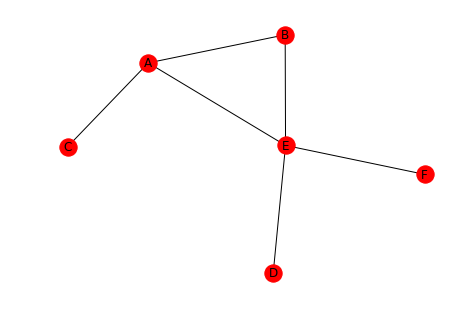In this graph there are six nodes so each node can have at most 5 links. E has 4 so the degree centrality should be 4/5.

degree_centrality = networkx.degree_centrality(graph)
print(degree_centrality)
assert degree_centrality["E"] == 4/5

{'B': 0.4, 'E': 0.8, 'A': 0.6000000000000001, 'C': 0.2, 'D': 0.2, 'F': 0.2}


## 3 Closeness Centrality

This measure assumes that the node that is closest to all the other nodes is the most important. It is the ratio of the highest possible degree for the node to the sum of the shortest paths to the other nodes.

\begin{equation*} Centrality_{closeness}(v) = \frac{|N| - 1}{\textit{sum of shortest paths of v}} \end{equation*}

Looking at E again, you can see that the sum of its shortest paths is 6 so the closeness centrality should be 5/6.

closeness_centrality = networkx.closeness_centrality(graph)
print(closeness_centrality)
assert closeness_centrality["E"] == 5/6

{'B': 0.625, 'E': 0.8333333333333334, 'A': 0.7142857142857143, 'C': 0.45454545454545453, 'D': 0.5, 'F': 0.5}


# Connected Components

# pypi
import networkx
from networkx import (
draw,
DiGraph,
Graph,
)

% matplotlib inline


## 1 Undirected Graphs

### 1.1 Connected Graphs

An unconnected graph is connected if every pair of nodes has a path between them.

left = tuple("AAAAABBCDFFFGGGHIJKKKLLLN")
right = tuple("BECGNCDDEGIJHIJIJOLMOMNOO")
undirected = Graph()
draw(undirected, with_labels=True)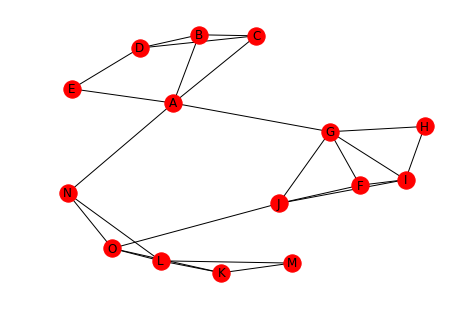Is this graph connected? It looks like it, since every node has an edge to it.

print(networkx.is_connected(undirected))

True


To make this graph unconnected you need to remove some edges that connect sub-graphs.

undirected.remove_edges_from([("A", "G"), ("A", "N")])
draw(undirected, with_labels=True)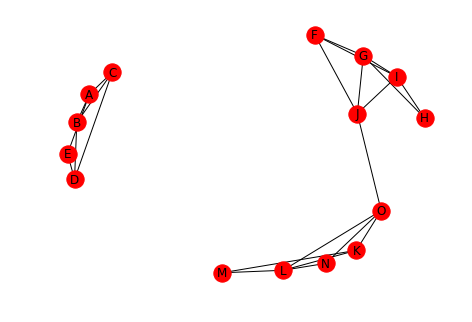print(networkx.is_connected(undirected))

False


### 1.2 Connected Components

A connected component is a subset of nodes where:

• Every node in the subset has a path to every other node
• No node outside the subset has a path to a node in the subset

Let's break the graph a little more.

undirected.remove_edge("J", "O")
draw(undirected, with_labels=True)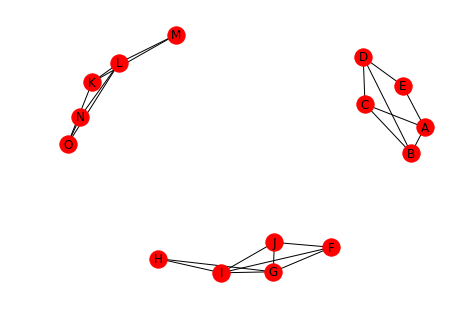We now have three connected components.

print(networkx.number_connected_components(undirected))

3


Which we can inspect.

for component in networkx.connected_components(undirected):
print(component)

{'J', 'I', 'G', 'H', 'F'}
{'N', 'M', 'O', 'K', 'L'}
{'D', 'B', 'C', 'E', 'A'}


We can also pick out a node from one of the components and get the sub-set.

print(networkx.node_connected_component(undirected, "A"))

{'B', 'D', 'C', 'A', 'E'}


Which you can see is the third connected component in the example above.

## 2 Directed Graphs

Directed graphs have similar ideas with regard to connectivity when compared to undirected graphs, but with a strong and weak version for each.

### 2.1 Strongly Connected

A strongly connected graph is a directed graph where for every pair of nodes there is a directed path in both directions.

left = tuple("AABCDD")
right = tuple("BDDBCA")

directed = DiGraph()
draw(directed, with_labels=True)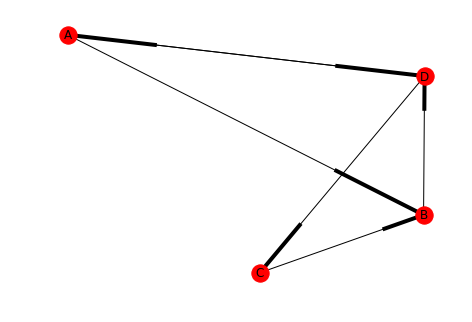For some reason networkx uses boxes instead of arrow-heads, but hopefully you get the idea.

print(networkx.is_strongly_connected(directed))

True


### 2.2 Weakly Connected

A directed graph is weakly connected if, when all the edges are replaced by undirected edges (converting it to an undirected graph) then the graph is connected.

directed.remove_edge("B", "D")
print(networkx.is_strongly_connected(directed))
print(networkx.is_weakly_connected(directed))

False
True

draw(directed, with_labels=True)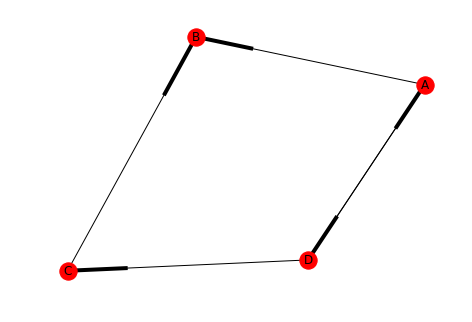Our new graph isn't strongly connected because there's no path from B to A (or B to C, etc.). But it is weakly connected since removing the directions just makes it a loop.

### 2.3 Strongly Connected Component

This is a subset of nodes in a directed graph where:

• Every node in the subset has a directed path to every other node
• No node outside the subset has a directed path to and from every node in the subset
left = tuple("ADDDEFFFFGGH")
right = tuple("HBFGGABGHHCE")
directed_2 = DiGraph()
draw(directed_2, with_labels=True)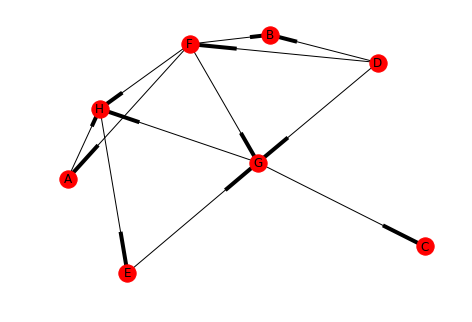print(networkx.is_strongly_connected(directed_2))

False


You can see that the graph is not strongly connected (there's no path to E, for instance) but is there a strongly connected component within it?

for component in networkx.strongly_connected_components(directed_2):
print(component)

{'C'}
{'H', 'G', 'E'}
{'A'}
{'B'}
{'F'}
{'D'}


In this case H, G, and E are a strongly connected component (as are each of the other individual nodes). What if we add a path from B to D?

directed_2.add_edge("B", "D")
draw(directed_2, with_labels=True)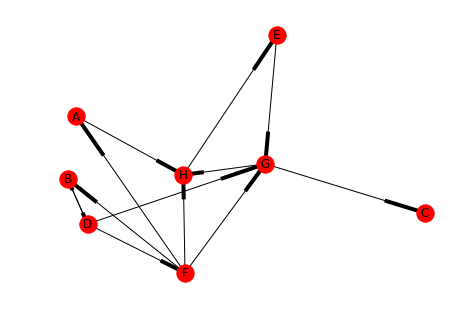for component in networkx.strongly_connected_components(directed_2):
print(component)

{'C'}
{'H', 'G', 'E'}
{'A'}
{'B', 'F', 'D'}


Now there are two interesting strongly connected components and two not so interesting ones.

### 2.4 Weakly Connected Components

A weakly connected component is one where a directed graph is converted into an undirected graph and the sub-set of nodes is a connected component.

directed_2.remove_edges_from([("F", "A"), ("F", "H"), ("F", "G"), ("D", "G"), ("B", "D"), ("E", "G")])
draw(directed_2, with_labels=True)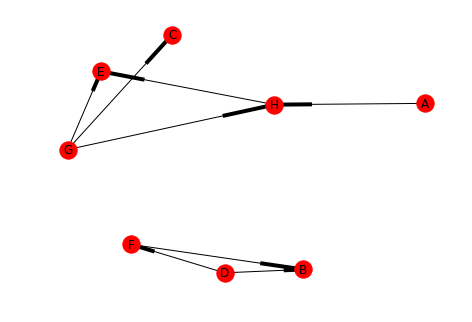for component in networkx.strongly_connected_components(directed_2):
print(component)

{'C'}
{'E'}
{'H'}
{'A'}
{'B'}
{'F'}
{'D'}
{'G'}

undirected_2 = directed_2.to_undirected()
draw(undirected_2, with_labels=True)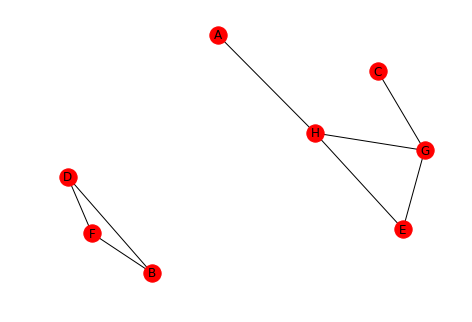Looking at the converted graph you can see that there are two connected components.

for component in networkx.connected_components(undirected_2):
print(component)

{'A', 'H', 'G', 'C', 'E'}
{'D', 'B', 'F'}


An important thing to note is that A and C are part of their connected component, even though visually they look like they're dangling out there.

You can also skip the conversion and let network x do it for you.

for component in networkx.weakly_connected_components(directed_2):
print(component)

{'G', 'C', 'H', 'E', 'A'}
{'B', 'F', 'D'}


# Distance in Social Networks

## 1 Introduction

When characterizing a graph one of the things to look at is how far apart the nodes are.

# from pypi
import networkx


This will be the example network.

left = tuple("AAKBCCFEDEIE")
right = tuple("KBBCFEGFEIJH")
graph = networkx.Graph()
networkx.draw(graph, with_labels=True)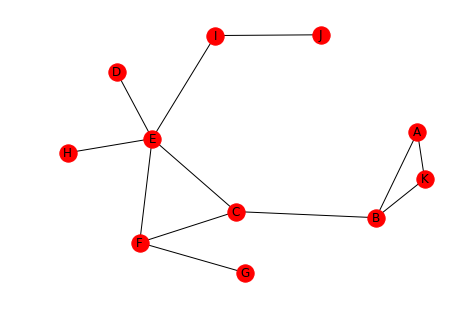## 2 Defining Distance

This section will look at how we can measure the distance between nodes.

### 2.1 Paths

A path is a sequence of nodes connected by edges. One path from D to K might be D-E-C-B-K.

left = tuple('DECB')
right = tuple("ECBK")
path = networkx.Graph()
networkx.draw(path, with_labels=True)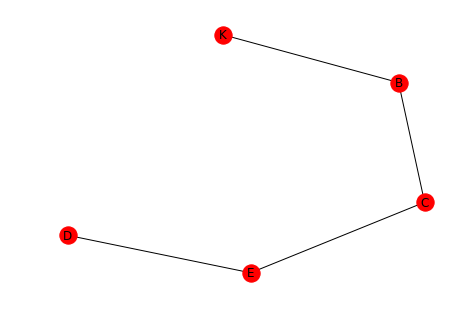### 2.2 Distance

• The length of a path is the number of edges in it.
• The distance between two nodes is the length of the shortest path between them.
dk_shortest_path = networkx.shortest_path(graph, "D", "K")
print(dk_shortest_path)

['D', 'E', 'C', 'B', 'K']

length = networkx.shortest_path_length(graph, "D", "K")
print(length)
assert len(dk_shortest_path) - 1 == networkx.shortest_path_length(graph, "D", "K")

4


As you can see the path we saw earlier is the shortest path and the distance from D to K is 4.

## 3 Graph Distance

This looks at how you can answer questions about the graph as a whole.

### 3.1 Average Distance

One measure is the average of the distances between ever pair of nodes.

print(networkx.average_shortest_path_length(graph))

2.5272727272727273


The average distance for our example is around two and a half edges.

### 3.2 Diameter

The diameter of a graph is the maximum distance between any of the pairs of nodes. Note that distance is always the shortest path between nodes, so this isn't the longest path in the graph.

print(networkx.diameter(graph))

5


The greatest distance is 5 hops in our example.

### 3.3 Eccentricity

This is the largest distance between a node and all the other nodes.

print(networkx.eccentricity(graph))

{'J': 5, 'D': 4, 'H': 4, 'F': 3, 'K': 5, 'A': 5, 'B': 4, 'C': 3, 'E': 3, 'G': 4, 'I': 4}


Looking at the output we can see that A, J, and K all have eccentricities matching the diameter. According to the Online Etymology Dictionary, eccentric means an orbiting object that doesn't have the earth at the center of its orbit. More literally, it means out of center (or off center).

The radius is the minimum eccentricity in a graph.

print(networkx.radius(graph))

3


So the radius is the smallest of the largest distances for all the nodes.

### 3.5 Periphery

This is the set of nodes whose eccentricity is equal to the diameter (5 in our case).

print(networkx.periphery(graph))

['J', 'K', 'A']


Looking at the output and the graph, the diameter of the graph is the distance from A to J or K to J.

### 3.6 Center

This is the set of nodes whose eccentricity is equal to the radius of the graph (3 in this example).

print(networkx.center(graph))

['F', 'C', 'E']

positions = networkx.drawing.nx_agraph.graphviz_layout(graph, prog="dot")
networkx.draw(graph, positions, with_labels=True)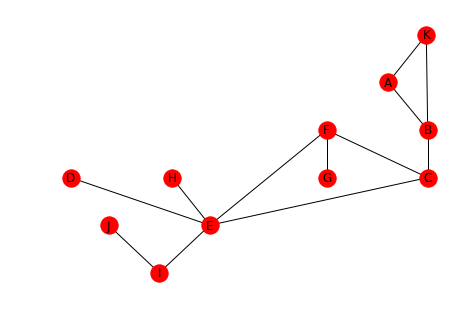Looking at the graph, you can see that F, C, and, E do in fact form the center triangle.

## 4 Karate Club

This looks at the network created by the relationships between members of a karate club that is on the verge of splitting up. Each node is a member of the club and the edges represent that the incident edges interacted with each other outside of the club (and were thus assumed to be friends). Members who didn't interact with each other outside of the club aren't represented in the data set.

The instructor wanted to raise fees while the officers didn't. Eventually the instructor was fired and his supporters left with him.

karate = networkx.karate_club_graph()
networkx.draw(karate, with_labels=True)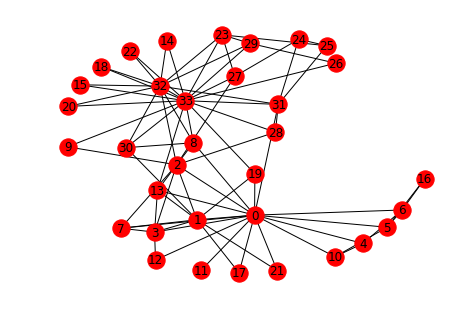networkx.draw_circular(karate, with_labels=True)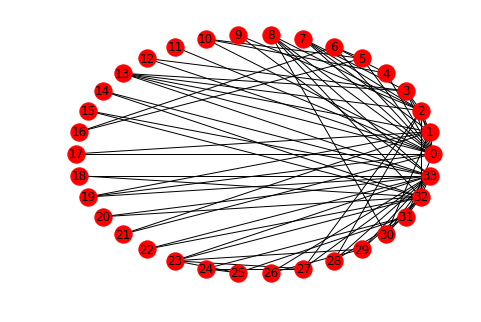You can see that there are some central characters in the club, notably 0, 32, and 33.

degrees = ((node, karate.degree(node)) for node in karate.nodes())
degrees = ((node, degree) for node, degree in degrees if degree > 10)
print("Node\tDegree")
for node, degree in degrees:
print("{}\t{}".format(node, degree))

Node Degree
0 16
32 12
33 17

The cut-off of 10 degrees was somewhat arbitrary, there are two nodes with degrees 9 and 10 respectively, but you can see that these three nodes were the most connected members of the club.

### 4.1 What is the average distance?

print(networkx.average_shortest_path_length(karate))

2.4


The path lengths are relatively short, on average.

### 4.2 Diameter

print(networkx.diameter(karate))

5


The maximum distance is 5.

### 4.3 Eccentricity

print(networkx.eccentricity(karate))

{0: 3, 1: 3, 2: 3, 3: 3, 4: 4, 5: 4, 6: 4, 7: 4, 8: 3, 9: 4, 10: 4, 11: 4, 12: 4, 13: 3, 14: 5, 15: 5, 16: 5, 17: 4, 18: 5, 19: 3, 20: 5, 21: 4, 22: 5, 23: 5, 24: 4, 25: 4, 26: 5, 27: 4, 28: 4, 29: 5, 30: 4, 31: 3, 32: 4, 33: 4}


What is the smallest eccentricity?

print(networkx.radius(karate))

3


### 4.5 Periphery

Which nodes are furthest apart?

print(networkx.periphery(karate))

[14, 15, 16, 18, 20, 22, 23, 26, 29]


### 4.6 Center

print(networkx.center(karate))

[0, 1, 2, 3, 8, 13, 19, 31]


The center nodes are most likely the ones that kept information flowing between the two factions (although node 0 is in here as well).

## Introduction

Triadic Closure is a measure of the tendency of edges in a graph to form triangles. It's a measure of the degree to which nodes in a graph tend to cluster together (wikipedia on clustering coefficents).

# python standard library
from fractions import Fraction

# pypi
import networkx
import seaborn

% matplotlib inline
seaborn.set_style("whitegrid")

sample_graph = networkx.Graph()
sample_graph.add_edges_from([(1, 2), (1, 3), (2, 4), (3, 5)])
networkx.draw_spring(sample_graph, with_labels=True)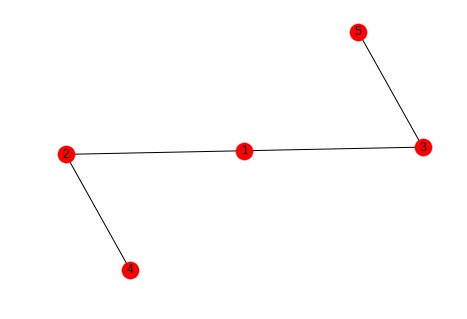In this case we might say that the likelihood that the next edge will be between 1 and 4 or 1 and 5 is greater than the likelihood that it will form between 4 and 5 or 2 and 5.

## 2 Local Clustering Coefficient

The Local Clustering Coefficient is a measure of clustering for a single node. It is the number of pairs of a node's friends that are themselves friends divided by the total number of pairs of a node's friends. This can be interpreted as measuring how close the node's neighbors are to being a complete graph (wikipedia).

\begin{equation*} LCC = \frac{\textit{number of pairs of a node's friends that are friends (PTAF)}}{\textit{number of pairs of the node's friends (POF)}} \end{equation*}
graph = networkx.Graph()
graph.add_edges_from([("A", "K"), ("A", "B"), ("A", "C"),
("B", "K"), ("B", "C"),
("C", "E"), ("C", "F"),
("D", "E"),
("E", "F"), ("E", "H"),
("F", "G"),
("I", "J")])

.. ggcode:: ipython

networkx.draw_spring(graph, with_labels=True)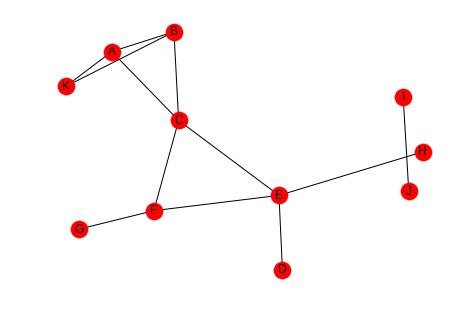The number of pairs of friends can be calculated from the degree of the node.

\begin{equation*} POF = \frac{d(d-1)}{2} \end{equation*}

Looking at node C, it has degree four so the number of pairs of friends it has is $$\frac{4(3)}{2} = 6$$. Looking at the graph you can see that there are two edges between the nodes connected to it - (A,B) and (E, F), so the clustering coefficient for node C is $$\frac{PTAF}{POF}=\frac{2}{6}$$ which reduces to 1/3. We can double check this with networkx.

print(networkx.clustering(graph, "C"))

0.3333333333333333


If you don't pass in the node label to networkx.clustering the function will return a dictionary with all the clustering coefficients, which might be useful if you need to make multiple queries and have a large graph.

### 2.1 One Friend

If you look at nodes I and J, they don't have any pairs of friends, just one friend each. This puts a zero in the denominator of the clustering coefficient, making it undefined, but to make it mathematically useful it is given a 0 instead.

print(networkx.clustering(graph, "I"))

0.0


## 3 The Whole Network

There's two ways to calculate a clustering coefficient for the entire network. One is to take the average of all the local clustering coefficients, the other is to calculate the percentage of open triads (three nodes connected by two edges) that are triangles.

### 3.1 Averaging

This is what wikipedia calls the network average clustering coefficient.

coefficients = networkx.clustering(graph)
average = sum(coefficients.values())/len(coefficients)
print(average)
assert average == networkx.average_clustering(graph)

0.28787878787878785


### 3.2 Transitivity

This is also called the global clustering coefficient.

A triangle is a set of three nodes with three edges connecting them. An open triad is a set of three nodes with only two edges connecting them. Each triangle has three open triads embedded in it. Transivity is a measure of the percentage of open triads that are triangles.

This triangle:

triangle = networkx.Graph()
triangle.add_edges_from([("A", "B"), ("A", "C"), ("B", "C")])

networkx.draw_spring(triangle, with_labels=True)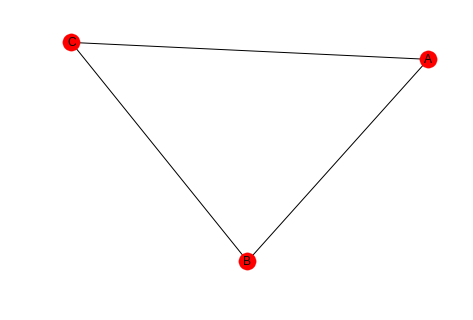one = networkx.Graph()
networkx.draw(one, with_labels=True)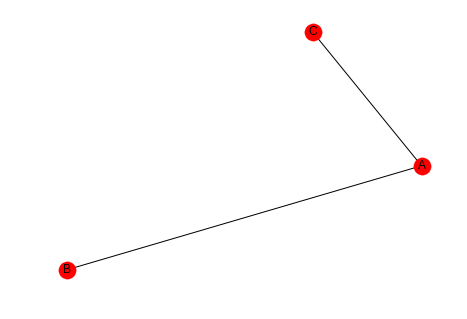two = networkx.Graph()
networkx.draw(two, with_labels=True)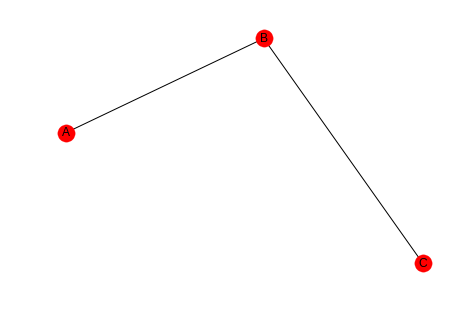three = networkx.Graph()
networkx.draw(three, with_labels=True)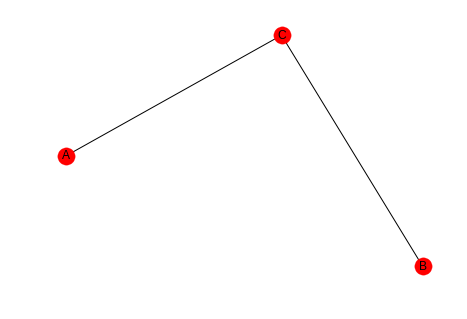So the transitivity is three times the count of triangles in the graph divided by all the open triads in the graph.

\begin{equation*} transitivity = \frac{3 \times \|\textit{triangles}\|}{\|\textit{open triads}\|} \end{equation*}

Looking at our earlier example you can see that there are three triangles and thirteen open triads (to be honest I only found 10).

networkx.draw_spring(graph, with_labels=True)transitivity = (3 * 3)/(3 * 3 + 13)
print(transitivity)
assert transitivity == networkx.transitivity(graph)

0.4090909090909091


## 4 Comparing Averaging and Transitivity

### 4.1 One High Degree Node

high_lcc = networkx.Graph()
left = tuple("AABCCDEEFGGH")
right = tuple("BIIDIIFIIHII")
networkx.draw_spring(high_lcc, with_labels=True)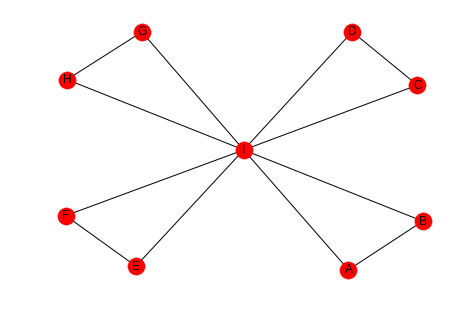If we look at this graph, the outer nodes all have a clustering coefficient of 1 (each has 1 pair of friends that are friends) while the center node has a coefficient of 1/7, since half the pairs don't have edges between them.

degree_i = 8
pairs_of_friends = Fraction(8 * 7, 2)
pairs_that_are_friends = Fraction(4, 1)
lcc = pairs_that_are_friends/pairs_of_friends
print(lcc)

1/7


Since there are so many nodes with a coefficient of 1, the average is high.

print(networkx.average_clustering(high_lcc))

0.9047619047619047


But there are many open triads so the transitivity will be low (transitivity weights nodes with large degree higher, but there's only one node with degree greater than 2).

print(networkx.transitivity(high_lcc))

0.3333333333333333


### 4.2 Many Open Pairs

outer_left = "ABDEGHJKMN"
inner_left = "PPPPQQQRRS"
outer_right = "BCEFHIKLNO"
inner_right = "QRSTRSTSTT"
left = tuple(outer_left + inner_left)
right = tuple(outer_right + inner_right)
low_average = networkx.Graph()
networkx.draw(low_average, with_labels=True)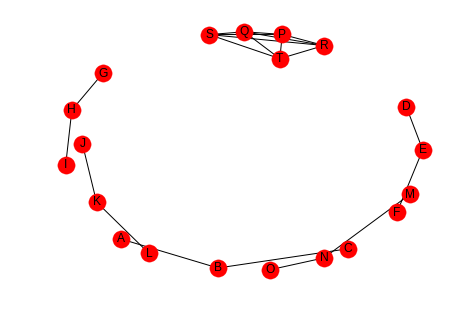Here the nodes P, Q, R, S, and T are completely connected (it's hard to see) but all the other nodes are open triads so the average will be low, but the transitivity will be high, because each of the P, Q, R, S, and T form triangles. This should be easier to see if they are plotted separately.

left = tuple(inner_left)
right = tuple(inner_right)
inner = networkx.Graph()
networkx.draw(inner, with_labels=True)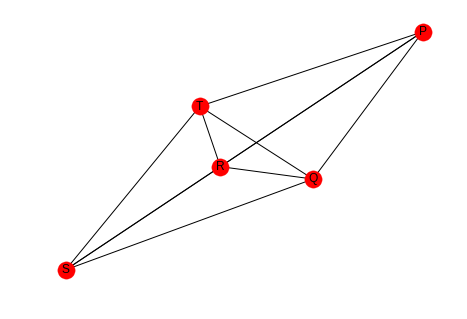Here's the average clustering coefficient (for the complete graph, not the sub-graph I just made).

print(networkx.average_clustering(low_average))

0.25


And here's the transitivity.

print(networkx.transitivity(low_average))

0.8571428571428571


So which one is the right metric? I guess it just depends.

# Assignment 2 - Introduction to NLTK

In part 1 of this assignment you will use nltk to explore the Herman Melville novel Moby Dick. Then in part 2 you will create a spelling recommender function that uses nltk to find words similar to the misspelling.

## 1 Part 1 - Analyzing Moby Dick

### 1.1 Imports and Data Set Up

from nltk.probability import FreqDist
from nltk.stem import WordNetLemmatizer
from nltk.tokenize import sent_tokenize
import matplotlib
import matplotlib.pyplot as pyplot
import nltk
import nltk.data
import numba
import numpy
import pandas
import seaborn

%matplotlib inline
seaborn.set_style("whitegrid")


If you would like to work with the raw text you can use 'moby_raw'.

with open('moby.txt', 'r') as reader:


If you would like to work with the novel in nltk.Text format you can use 'text1'.

moby_tokens = nltk.word_tokenize(moby_raw)
text = nltk.Text(moby_tokens)
moby_series = pandas.Series(moby_tokens)


### 1.2 Examples

#### 1.2.1 Example 1

How many tokens (words and punctuation symbols) are in text1? A token is a linguistic unit such as a word, punctuation mark, or alpha-numeric strings.

This function should return an integer.

def example_one():
"""counts the tokens in moby dick

Returns:
int: number of tokens in moby dick
"""
# or alternatively len(text1)
return len(moby_tokens)

MOBY_TOKEN_COUNT = example_one()
print("Moby Dick has {:,} tokens.".format(
MOBY_TOKEN_COUNT))

Moby Dick has 254,989 tokens.


#### 1.2.2 Example 2

How many unique tokens (unique words and punctuation) does text1 have?

This function should return an integer.

def example_two():
"""counts the unique tokens

Returns:
int: count of unique tokens in Moby Dick
"""
# or alternatively len(set(text1))
return len(set(nltk.word_tokenize(moby_raw)))

MOBY_UNIQUE_COUNT = example_two()
print("Moby Dick has {:,} unique tokens.".format(
MOBY_UNIQUE_COUNT))

Moby Dick has 20,755 unique tokens.


#### 1.2.3 Example 3

After lemmatizing the verbs, how many unique tokens does text1 have? A lemma is the canonical form. e.g. run is the lemma for runs, ran, running, and run.

This function should return an integer.

def example_three():
"""Counts the number of lemma in Moby Dick

Returns:
int: count of unique lemma
"""
lemmatizer = WordNetLemmatizer()
return len(set([lemmatizer.lemmatize(w,'v') for w in text1]))

MOBY_LEMMA_COUNT = example_three()
print("Moby Dick has {:,} lemma (found in WordNet).".format(
MOBY_LEMMA_COUNT))

Moby Dick has 16,900 lemma (found in WordNet).


### 1.3 Questions

#### 1.3.1 Question 1

What is the lexical diversity of the given text input? (i.e. ratio of unique tokens to the total number of tokens)

This function should return a float.

@jit
def lexical_diversity(tokens):
"""Calculates the lexical diversity of a list of tokens

Returns:
float: fraction of tokens that are unique
"""
return len(set(tokens))/float(len(tokens))

def answer_one():
"""Calculates the lexical diversity of Moby Dick

Returns:
float: fraction of tokens that are unique
"""
return lexical_diversity(moby_tokens)

print("Lexical Diversity of Moby Dick: {:.2f}".format(output))

Lexical Diversity of Moby Dick: 0.08


About 8 percent of the tokens in Moby Dick are unique.

#### 1.3.2 Question 2

What percentage of tokens is 'whale'or 'Whale'?

This function should return a float.

moby_frequencies = FreqDist(moby_tokens)

def answer_two():
"""calculates percentage of tokens that are 'whale'

Returns:
float: percentage of entries that are whales
"""
whales = moby_frequencies["whale"] + moby_frequencies["Whale"]
return 100 * (whales/float(MOBY_TOKEN_COUNT))

print("Percentage of tokens that are whales: {:.2f} %".format(whale_fraction))

Percentage of tokens that are whales: 0.41 %


Around 1 percent of the tokens are 'whale'.

I originally made two mistakes with this question, I was returning a fraction, not a percentage, and I was using a regular expression '([Ww]hale)' which I later realized would match whales, whaler, and other variants.

782


#### 1.3.3 Question 3

What are the 20 most frequently occurring (unique) tokens in the text? What is their frequency?

This function should return a list of 20 tuples where each tuple is of the form (token, frequency). The list should be sorted in descending order of frequency.

def answer_three():
"""finds 20 most requently occuring tokens

Returns:
list: (token, frequency) for top 20 tokens
"""
return moby_frequencies.most_common(20)


[(',', 19204), ('the', 13715), ('.', 7308), ('of', 6513), ('and', 6010), ('a', 4545), ('to', 4515), (';', 4173), ('in', 3908), ('that', 2978), ('his', 2459), ('it', 2196), ('I', 2097), ('!', 1767), ('is', 1722), ('--', 1713), ('with', 1659), ('he', 1658), ('was', 1639), ('as', 1620)]


#### 1.3.4 Question 4

What tokens have a length of greater than 5 and frequency of more than 150?

This function should return a sorted list of the tokens that match the above constraints. To sort your list, use sorted()

moby_frequency_frame = pandas.DataFrame(moby_frequencies.most_common(),
columns=["token", "frequency"])

def answer_four():
"""gets tokens with length > 5, frequency > 150"""
frame =  moby_frequency_frame[(moby_frequency_frame.frequency > 150)
& (moby_frequency_frame.token.str.len() > 5)]
return sorted(frame.token)

print(output)

['Captain', 'Pequod', 'Queequeg', 'Starbuck', 'almost', 'before', 'himself', 'little', 'seemed', 'should', 'though', 'through', 'whales', 'without']


I was originally returning the data frame, not just the sorted tokens, which was of course marked wrong.

#### 1.3.5 Question 5

Find the longest word in text1 and that word's length.

This function should return a tuple (longest_word, length).

def answer_five():
"""finds the longest word and its length

Return:
tuple: (longest-word, length)
"""
length = max(moby_frequency_frame.token.str.len())
longest = moby_frequency_frame.token.str.extractall("(?P<long>.{{{}}})".format(length))
return (longest.long.iloc, length)


("twelve-o'clock-at-night", 23)


#### 1.3.6 Question 6

What unique words have a frequency of more than 2000? What is their frequency?

Hint: you may want to use isalpha() to check if the token is a word and not punctuation.

This function should return a list of tuples of the form (frequency, word) sorted in descending order of frequency.

moby_words = moby_frequency_frame[moby_frequency_frame.token.str.isalpha()]

def answer_six():
"""Finds words wih frequency > 2000

Returns:
list: frequency, word tuples
"""
common = moby_words[moby_words.frequency > 2000]
return list(zip(common.frequency, common.token))


[(13715, 'the'), (6513, 'of'), (6010, 'and'), (4545, 'a'), (4515, 'to'), (3908, 'in'), (2978, 'that'), (2459, 'his'), (2196, 'it'), (2097, 'I')]


When I first submitted this I got it wrong because I was returning a list of (word, frequency), not (frequency, word).

#### 1.3.7 Question 7

What is the average number of tokens per sentence?

This function should return a float.

def answer_seven():
"""average number of tokens per sentence"""
sentences = sent_tokenize(moby_raw)
counts = (len(nltk.word_tokenize(sentence)) for sentence in sentences)
return sum(counts)/float(len(sentences))

print("Average number of tokens per sentence: {:.2f}".format(output))

Average number of tokens per sentence: 25.88


#### 1.3.8 Question 8

What are the 5 most frequent parts of speech in this text? What is their frequency? Parts of Speech (POS) are the lexical categories that words belong to.

This function should return a list of tuples of the form (part_of_speech, frequency) sorted in descending order of frequency.

def answer_eight():
"""gets the 5 most frequent parts of speech

Returns:
list (Tuple): (part of speech, frequency) for top 5
"""
tags = nltk.pos_tag(moby_words.token)
frequencies = FreqDist([tag for (word, tag) in tags])
return frequencies.most_common(5)

print("Top 5 parts of speech: {}".format(output))

Top 5 parts of speech: [('NN', 4016), ('NNP', 2916), ('JJ', 2875), ('NNS', 2452), ('VBD', 1421)]


## 2 Part 2 - Spelling Recommender

For this part of the assignment you will create three different spelling recommenders, that each take a list of misspelled words and recommends a correctly spelled word for every word in the list.

For every misspelled word, the recommender should find find the word in correct_spellings that has the shortest distance*, and starts with the same letter as the misspelled word, and return that word as a recommendation.

Each of the three different recommenders will use a different distance measure (outlined below).

Each of the recommenders should provide recommendations for the three default words provided: ['cormulent', 'incendenece', 'validrate'].

from nltk.corpus import words
from nltk.metrics.distance import (
edit_distance,
jaccard_distance,
)
from nltk.util import ngrams

correct_spellings = words.words()
spellings_series = pandas.Series(correct_spellings)


### 2.1 Question 9

For this recommender, your function should provide recommendations for the three default words provided above using the following distance metric:

Jaccard distance on the trigrams of the two words.

This function should return a list of length three: ['cormulent_reccomendation', 'incendenece_reccomendation', 'validrate_reccomendation'].

def jaccard(entries, gram_number):
"""find the closet words to each entry

Args:
entries: collection of words to match
gram_number: number of n-grams to use

Returns:
list: words with the closest jaccard distance to entries
"""
outcomes = []
for entry in entries:
spellings = spellings_series[spellings_series.str.startswith(entry)]
distances = ((jaccard_distance(set(ngrams(entry, gram_number)),
set(ngrams(word, gram_number))), word)
for word in spellings)
closest = min(distances)
outcomes.append(closest)
return outcomes

def answer_nine(entries=['cormulent', 'incendenece', 'validrate']):
"""finds the closest word based on jaccard distance"""
return jaccard(entries, 3)


['corpulent', 'indecence', 'validate']


I originally got both the Jaccard Distance problems wrong because I was just using the distance, not filtering the candidates by the first letter, which turns out to return fairly dissimilar words.

### 2.2 Question 10

For this recommender, your function should provide recommendations for the three default words provided above using the following distance metric:

Jaccard distance on the 4-grams of the two words.

This function should return a list of length three: ['cormulent_reccomendation', 'incendenece_reccomendation', 'validrate_reccomendation'].

def answer_ten(entries=['cormulent', 'incendenece', 'validrate']):
"""gets the neares words using jaccard-distance with 4-grams

Args:
entries (list): words to find nearest other word for

Returns:
list: nearest words found
"""
return jaccard(entries, 4)


['cormus', 'incendiary', 'valid']


### 2.3 Question 11

For this recommender, your function should provide recommendations for the three default words provided above using the following distance metric:

Edit (Levenshtein) distance on the two words with transpositions.

This function should return a list of length three: ['cormulent_reccomendation', 'incendenece_reccomendation', 'validrate_reccomendation'].

def answer_eleven(entries=['cormulent', 'incendenece', 'validrate']):
"""gets the nearest words based on Levenshtein distance

Args:
entries (list[str]): words to find closest words to

Returns:
list[str]: nearest words to the entries
"""
outcomes = []
for entry in entries:
distances = ((edit_distance(entry,
word), word)
for word in correct_spellings)
closest = min(distances)
outcomes.append(closest)
return outcomes


['corpulent', 'intendence', 'validate']


# Extracting Dates From Medical Data

## 1 Introduction

In this assignment, you'll be working with messy medical data and using regular expressions to extract relevant information from the data.

Each line of the dates.txt file corresponds to a medical note. Each note has a date that needs to be extracted, but each date is encoded in one of many formats.

The goal of this assignment is to correctly identify all of the different date variants encoded in this dataset and to properly normalize and sort the dates.

Here is a list of some of the variants you might encounter in this dataset:

• 04/20/2009; 04/20/09; 4/20/09; 4/3/09
• Mar-20-2009; Mar 20, 2009; March 20, 2009; Mar. 20, 2009; Mar 20 2009;
• 20 Mar 2009; 20 March 2009; 20 Mar. 2009; 20 March, 2009
• Mar 20th, 2009; Mar 21st, 2009; Mar 22nd, 2009
• Feb 2009; Sep 2009; Oct 2010
• 6/2008; 12/2009
• 2009; 2010

Once you have extracted these date patterns from the text, the next step is to sort them in ascending chronological order accoring to the following rules:

• Assume all dates in xx/xx/xx format are mm/dd/yy
• Assume all dates where year is encoded in only two digits are years from the 1900's (e.g. 1/5/89 is January 5th, 1989)
• If the day is missing (e.g. 9/2009), assume it is the first day of the month (e.g. September 1, 2009).
• If the month is missing (e.g. 2010), assume it is the first of January of that year (e.g. January 1, 2010).

With these rules in mind, find the correct date in each note and return a pandas Series in chronological order of the original Series' indices.

0    1999
1    2010
2    1978
3    2015
4    1985

0    2
1    4
2    0
3    1
4    3


Your score will be calculated using Kendall's tau, a correlation measure for ordinal data.

This function should return a Series of length 500 and dtype int.

## 2 Imports

# from pypi
import pandas


with open('dates.txt') as reader:


0         03/25/93 Total time of visit (in minutes):\n
1                       6/18/85 Primary Care Doctor:\n
2    sshe plans to move as of 7/8/71 In-Home Servic...
3                7 on 9/27/75 Audit C Score Current:\n
4    2/6/96 sleep studyPain Treatment Pain Level (N...
5                    .Per 7/06/79 Movement D/O note:\n
6    4, 5/18/78 Patient's thoughts about current su...
7    10/24/89 CPT Code: 90801 - Psychiatric Diagnos...
8                         3/7/86 SOS-10 Total Score:\n
9             (4/10/71)Score-1Audit C Score Current:\n
dtype: object

data.describe()

count                                                   500
unique                                                  500
top       sApproximately 7 psychiatric hospitalizations ...
freq                                                      1
dtype: object


## 4 The Grammar

### 4.1 Cardinality

ZERO_OR_MORE = '*'
ONE_OR_MORE = "+"
ZERO_OR_ONE = '?'
EXACTLY_TWO = "{2}"
ONE_OR_TWO = "{1,2}"
EXACTLY_ONE = '{1}'


### 4.2 Groups and Classes

GROUP = r"({})"
NAMED = r"(?P<{}>{})"
CLASS = "[{}]"
NEGATIVE_LOOKBEHIND = "(?<!{})"
POSITIVE_LOOKBEHIND = "(?<={})"
ESCAPE = "\{}"


### 4.3 Numbers

DIGIT = r"\d"
ONE_DIGIT  = DIGIT + EXACTLY_ONE
ONE_OR_TWO_DIGITS = DIGIT + ONE_OR_TWO
TWO_DIGITS = DIGIT + EXACTLY_TWO
THREE_DIGITS = DIGIT + "{3}"
EXACTLY_TWO_DIGITS = DIGIT + EXACTLY_TWO + NON_DIGIT
FOUR_DIGITS = DIGIT + r"{4}" + NON_DIGIT


### 4.4 String Literals

SLASH = r"/"
OR = r'|'
LOWER_CASE = "a-z"
SPACE = "\s"
DOT = "."
DASH = "-"
COMMA = ","
PUNCTUATION = CLASS.format(DOT + COMMA + DASH)
EMPTY_STRING = ""


### 4.5 Dates

These are parts to build up the date-expressions.

MONTH_SUFFIX = (CLASS.format(LOWER_CASE) + ZERO_OR_MORE
+ CLASS.format(SPACE + DOT + COMMA + DASH) + ONE_OR_TWO)
MONTH_PREFIXES = "Jan Feb Mar Apr May Jun Jul Aug Sep Oct Nov Dec".split()
MONTHS = [month + MONTH_SUFFIX for month in MONTH_PREFIXES]
MONTHS = GROUP.format(OR.join(MONTHS))
DAY_SUFFIX = CLASS.format(DASH + COMMA + SPACE) + ONE_OR_TWO
DAYS = ONE_OR_TWO_DIGITS + DAY_SUFFIX
YEAR = FOUR_DIGITS


This is for dates like Mar 21st, 2009, those with suffixes on the days.

CONTRACTED = (ONE_OR_TWO_DIGITS
+ LOWER_CASE
+ EXACTLY_TWO
)
CONTRACTION = NAMED.format("contraction",
MONTHS
+ CONTRACTED
+ DAY_SUFFIX
+ YEAR)


This is for dates that have no days in them, like May 2009.

NO_DAY_BEHIND = NEGATIVE_LOOKBEHIND.format(DIGIT + SPACE)
NO_DAY = NAMED.format("no_day", NO_DAY_BEHIND + MONTHS + YEAR)


This is for the most common form (that I use) - May 21, 2017.

WORDS = NAMED.format("words", MONTHS + DAYS + YEAR)


This is for the case where the day is placed before them month - 20 March, 2009.

BACKWARDS = NAMED.format("backwards", ONE_OR_TWO_DIGITS + SPACE + MONTHS + YEAR)


This is the case where slashes are used but only two digits were used for the year (so we're assuming it's in the twentieth century) - 8/4/98.

slashed = SLASH.join([ONE_OR_TWO_DIGITS,
ONE_OR_TWO_DIGITS,
EXACTLY_TWO_DIGITS])
dashed = DASH.join([ONE_OR_TWO_DIGITS,
ONE_OR_TWO_DIGITS,
EXACTLY_TWO_DIGITS])
TWENTIETH_CENTURY = NAMED.format("twentieth",
OR.join([slashed, dashed]))


This is the case where digits with slashes are used and all four digits are used for the year - 8/4/1998.

NUMERIC = NAMED.format("numeric",
SLASH.join([ONE_OR_TWO_DIGITS,
ONE_OR_TWO_DIGITS,
FOUR_DIGITS]))


This is the case where only month and year are given as digits - 9/2009. There are two expressions, because the day can be one or two digits.

NO_PRECEDING_SLASH = NEGATIVE_LOOKBEHIND.format(SLASH)
NO_PRECEDING_SLASH_DIGIT = NEGATIVE_LOOKBEHIND.format(CLASS.format(SLASH + DIGIT))
NO_ONE_DAY = (NO_PRECEDING_SLASH_DIGIT
+ ONE_DIGIT
+ SLASH
+ FOUR_DIGITS)
NO_TWO_DAYS = (NO_PRECEDING_SLASH
+ TWO_DIGITS
+ SLASH
+ FOUR_DIGITS)
NO_DAY_NUMERIC = NAMED.format("no_day_numeric",
NO_ONE_DAY
+ OR
+ NO_TWO_DAYS
)


This is the case where only a year was given. This is the hardest case, since you don't want to accidentally match the other cases, but the text preceding and following it could be anything. For the look-behind, all the cases have to have the same number of characters so we can't re-use the other expressions

CENTURY = GROUP.format('19' + OR + "20") + TWO_DIGITS
DIGIT_SLASH = DIGIT + SLASH
DIGIT_DASH = DIGIT + DASH
DIGIT_SPACE = DIGIT + SPACE
LETTER_SPACE = CLASS.format(LOWER_CASE) + SPACE
COMMA_SPACE = COMMA + SPACE
YEAR_PREFIX = NEGATIVE_LOOKBEHIND.format(OR.join([
DIGIT_SLASH,
DIGIT_DASH,
DIGIT_SPACE,
LETTER_SPACE,
COMMA_SPACE,
]))

YEAR_ONLY = NAMED.format("year_only",
YEAR_PREFIX + CENTURY
)


These are leftovers that don't really match anything.

IN_PREFIX = POSITIVE_LOOKBEHIND.format(CLASS.format('iI') + 'n' + SPACE) + CENTURY
SINCE_PREFIX = POSITIVE_LOOKBEHIND.format(CLASS.format("Ss") + 'ince' + SPACE) + CENTURY
AGE = POSITIVE_LOOKBEHIND.format("Age" + SPACE + TWO_DIGITS + COMMA + SPACE) + CENTURY
AGE_COMMA = POSITIVE_LOOKBEHIND.format("Age" + COMMA + SPACE + TWO_DIGITS + COMMA + SPACE) + CENTURY
OTHERS = ['delivery', "quit", "attempt", "nephrectomy", THREE_DIGITS]
OTHERS = [POSITIVE_LOOKBEHIND.format(label + SPACE) + CENTURY for label in OTHERS]
OTHERS = OR.join(OTHERS)
LEFTOVERS_PREFIX = OR.join([IN_PREFIX, SINCE_PREFIX, AGE, AGE_COMMA]) + OR + OTHERS
LEFTOVERS = NAMED.format("leftovers", LEFTOVERS_PREFIX)


This is the combined expression for all the dates - the one that should be used to extract them from the data.

DATE = NAMED.format("date", OR.join([NUMERIC,
TWENTIETH_CENTURY,
WORDS,
BACKWARDS,
CONTRACTION,
NO_DAY,
NO_DAY_NUMERIC,
YEAR_ONLY,
LEFTOVERS]))

def twentieth_century(date):
"""adds a 19 to the year

Args:
date (re.Regex): Extracted date
"""
month, day, year = date.group(1).split(SLASH)
year = "19{}".format(year)
return SLASH.join([month, day, year])

def take_two(line):
match = re.search(TWENTIETH_CENTURY, line)
if match:
return twentieth_century(match)
return line


## 5 Applying The Grammer

def extract_and_count(expression, data, name):
"""extract all matches and report the count

Args:
expression (str): regular expression to match
data (pandas.Series): data with dates to extratc
name (str): name of the group for the expression

Returns:
tuple (pandas.Series, int): extracted dates, count
"""
extracted = data.str.extractall(expression)[name]
count = len(extracted)
print("'{}' matched {} rows".format(name, count))
return extracted, count

numeric, numeric_count = extract_and_count(NUMERIC, data, 'numeric')

'numeric' matched 25 rows

twentieth, twentieth_count = extract_and_count(TWENTIETH_CENTURY, data, 'twentieth')

'twentieth' matched 100 rows

words, words_count = extract_and_count(WORDS, data, 'words')

'words' matched 34 rows

backwards, backwards_count = extract_and_count(BACKWARDS, data, 'backwards')

'backwards' matched 69 rows

contraction_data, contraction = extract_and_count(CONTRACTION, data, 'contraction')

'contraction' matched 0 rows

no_day, no_day_count = extract_and_count(NO_DAY, data, 'no_day')

'no_day' matched 115 rows

no_day_numeric, no_day_numeric_count = extract_and_count(NO_DAY_NUMERIC, data,
"no_day_numeric")

'no_day_numeric' matched 112 rows

year_only, year_only_count = extract_and_count(YEAR_ONLY, data, "year_only")

'year_only' matched 15 rows

leftovers, leftovers_count = extract_and_count(LEFTOVERS, data, "leftovers")

'leftovers' matched 30 rows

found = data.str.extractall(DATE)
total_found = len(found.date)

print("Total Found: {}".format(total_found))
print("Remaining: {}".format(len(data) - total_found))
print("Discrepancy: {}".format(total_found - (numeric_count
+ twentieth_count
+ words_count
+ backwards_count
+ contraction
+ no_day_count
+ no_day_numeric_count
+ year_only_count
+ leftovers_count)))

Total Found: 500
Remaining: 0
Discrepancy: 0

missing = [label for label in data.index if label not in found.index.levels]
try:
print(missing, data.loc[missing])
except IndexError:
print("all rows matched")

all rows matched


## 6 Unifying the Formats

To make it simpler, I'm going to use the mm/dd/yyyy format for the dates. I'm going to use the extracted series to avoid having different clean-up cases contaminating each other - e.g. dealing with 'January' when the day comes first as opposed to when the month comes first.

### 6.1 Helper Functions

#### 6.1.1 Clean

This is a generic function to clean up some data. I was initially using it directly, but for cases where the expression and replacement function are used more than once, there are helper functions to make it easier.

def clean(source, expression, replacement, sample=5):
"""applies the replacement to the source

as a side-effect shows sample rows before and after

Args:
source (pandas.Series): source of the strings
expression (str): regular expression to match what to replace
replacement: function or expression to replace the matching expression
sample (int): number of randomly chosen examples to show

Returns:
pandas.Series: the source with the replacement applied to it
"""
print("Random Sample Before:")
print(source.sample(sample))
cleaned = source.str.replace(expression, replacement)
print("\nRandom Sample After:")
print(cleaned.sample(sample))
print("\nCount of cleaned: {}".format(len(cleaned)))
assert len(source) == len(cleaned)
return cleaned


#### 6.1.2 Clean Punctuation

def clean_punctuation(source, sample=5):
"""removes punctuation

Args:
source (pandas.Series): data to clean
sample (int): size of sample to show

Returns:
pandas.Series: source with punctuation removed
"""
print("Cleaning Punctuation")
if any(source.str.contains(PUNCTUATION)):
source = clean(source, PUNCTUATION, EMPTY_STRING)
return source


#### 6.1.3 Convert Long Month Names to Three-Letter Names

LONG_TO_SHORT = dict(January="Jan",
February="Feb",
March="Mar",
April="Apr",
May="May",
June="Jun",
July="Jul",
August="Aug",
September="Sep",
October="Oct",
November="Nov",
December="Dec")

# it turns out there are spelling errors in the data so this has to be fuzzy
LONG_TO_SHORT_EXPRESSION = OR.join([GROUP.format(month)
+ CLASS.format(LOWER_CASE)
+ ZERO_OR_MORE
for month in LONG_TO_SHORT.values()])

def long_month_to_short(match):
"""convert long month to short

Args:
match (re.Match): object matching a long month

Returns:
str: shortened version of the month
"""
return match.group(match.lastindex)


This next function is the one you would actually use to make the conversion.

def convert_long_months_to_short(source, sample=5):
"""convert long month names to short

Args:
source (pandas.Series): data with months
sample (int): size of sample to show

Returns:
pandas.Series: data with short months
"""
return clean(source,
LONG_TO_SHORT_EXPRESSION,
long_month_to_short)


#### 6.1.4 Add January 1 to year-only dates

def add_month_date(match):

Args:
match (re.Match): object that only matched a 4-digit year

Returns:
str: 01/01/YYYY
"""
return "01/01/" + match.group()


And now the function to actually call.

def add_january_one(source):

Args:
source (pandas.Series): data with the dates

Returns:
pandas.Series: years in source with /01/01/ added
"""


#### 6.1.5 Two-Digit Numbers

This makes sure that there are exactly two digits in a number, adding a leading zero if needed.

two_digit_expression = GROUP.format(ONE_OR_TWO_DIGITS) + POSITIVE_LOOKAHEAD.format(SLASH)

def two_digits(match):

Args:
match (re.Match): match with one or two digits

Returns:
str: the matched string with leading zero if needed
"""
# for some reason the string-formatting raises an error if it's a string
# so cast it to an int
return "{:02}".format(int(match.group()))


This is the function to call for the case where the number is followed by a slash (e.g. 2/).

def clean_two_digits(source, sample=5):
"""makes sure source has two-digits

Args:
source (pandas.Series): data with digit followed by slash
sample (int): number of samples to show

Returns:
pandas.Series: source with digits coerced to two digits
"""
return clean(source, two_digit_expression, two_digits, sample)


This is like clean_two_digits but it doesn't check for the trailing slash. Use this if you have an isolated column of numbers that need to be two-digits.

def clean_two_digits_isolated(source, sample=5):
"""cleans two digits that are standalone

Args:
source (pandas.Series): source of the data
sample (int): number of samples to show

Returns:
pandas.Series: converted data
"""
return clean(source, ONE_OR_TWO_DIGITS, two_digits, sample)


#### 6.1.6 Cleaning Up Months

These clean up and convert written months (e.g. change Aug to 08).

digits = ("{:02}".format(month) for month in range(1, 13))
MONTH_TO_DIGITS = dict(zip(MONTH_PREFIXES, digits))
SHORT_MONTHS_EXPRESSION = OR.join((GROUP.format(month) for month in MONTH_TO_DIGITS))
def month_to_digits(match):
"""converts short month to digits

Args:
match (re.Match): object with short-month

Returns:
str: month as two-digit number (e.g. Jan -> 01)
"""
return MONTH_TO_DIGITS[match.group()]

def convert_short_month_to_digits(source, sample=5):
"""converts three-letter months to two-digits

Args:
source (pandas.Series): data with three-letter months
sample (int): number of samples to show

Returns:
pandas.Series: source with short-months coverted to digits
"""
return clean(source,
SHORT_MONTHS_EXPRESSION,
month_to_digits,
sample)


This function runs the previous three and is the main one that should be used. The others can be run individually for troubleshooting, though.

def clean_months(source, sample=5):
"""clean up months (which start as words)

Args:
source (pandas.Series): source of the months
sample (int): number of random samples to show
"""
cleaned = clean_punctuation(source)

print("Converting long months to short")
cleaned = clean(cleaned,
LONG_TO_SHORT_EXPRESSION,
long_month_to_short, sample)

print("Converting short months to digits")
cleaned = clean(cleaned,
SHORT_MONTHS_EXPRESSION,
month_to_digits, sample)
return cleaned


#### 6.1.7 Frame To Series

This is for the case where the date-fields were broken up into columns in a data-frame.

def frame_to_series(frame, index_source, samples=5):
"""re-combines data-frame into a series

Args:
frame (pandas.DataFrame): frame with month, day, year columns
index_source (pandas.series): source to copy index from
samples (index): number of random entries to print when done

Returns:
pandas.Series: series with dates as month/day/year
"""
combined = frame.month + SLASH + frame.day + SLASH + frame.year
combined.index = index_source.index
print(combined.sample(samples))
return combined


### 6.2 Year Only

For the case where there is only a year, I'll add January 1 to the dates.

year_only_cleaned = add_january_one(year_only)

Random Sample Before:
match
472  0        2010
495  0        1979
497  0        2008
481  0        1974
486  0        1973
Name: year_only, dtype: object

Random Sample After:
match
495  0        01/01/1979
470  0        01/01/1983
462  0        01/01/1988
481  0        01/01/1974
480  0        01/01/2013
Name: year_only, dtype: object

Count of cleaned: 15


### 6.3 Leftovers

These were the odd cases that didn't seem to have a real pattern. Since I used a positive lookbehind to match everything but the year they only have the years in them, like the previous year-only cases.

leftovers_cleaned = add_january_one(leftovers)

Random Sample Before:
match
487  0        1992
477  0        1994
498  0        2005
488  0        1977
484  0        2004
Name: leftovers, dtype: object

Random Sample After:
match
464  0        01/01/2016
455  0        01/01/1984
465  0        01/01/1976
475  0        01/01/2015
498  0        01/01/2005
Name: leftovers, dtype: object

Count of cleaned: 30

cleaned = pandas.concat([year_only_cleaned, leftovers_cleaned])
print(len(cleaned))

45


### 6.4 No Day Numeric

This is for the case where the date is formatted with slashes and there are no day-values. To make the months uniform I'm going to make them all two-digits first.

no_day_numeric_cleaned = clean_two_digits(no_day_numeric)

Random Sample Before:
match
450  0         1/1994
374  0        11/2000
403  0        10/1981
454  0         7/1982
358  0         1/1983
Name: no_day_numeric, dtype: object

Random Sample After:
match
426  0        11/1984
415  0        02/1973
360  0        12/2008
367  0        09/2001
362  0        08/2003
Name: no_day_numeric, dtype: object

Count of cleaned: 112


no_day_numeric_cleaned = clean(no_day_numeric_cleaned,
SLASH,
lambda m: "/01/")

Random Sample Before:
match
368  0        08/1986
409  0        10/1994
443  0        09/2000
404  0        10/1986
395  0        02/1977
Name: no_day_numeric, dtype: object

Random Sample After:
match
349  0        05/01/1987
392  0        05/01/2000
448  0        05/01/2010
394  0        10/01/2001
424  0        04/01/1979
Name: no_day_numeric, dtype: object

Count of cleaned: 112


And add it to the total.

original = len(cleaned)
cleaned = pandas.concat([cleaned, no_day_numeric_cleaned])
assert len(cleaned) == no_day_numeric_count + original

print(len(cleaned))

157


### 6.5 No Day

This is for cases like Mar 2011 where no day was given. We're going to assume that it's the first day of the month for each case.

no_day_cleaned = clean_months(no_day)

Cleaning Punctuation
Random Sample Before:
match
261  0           Oct 1986
269  0          July 1992
280  0          July 1985
295  0         March 1983
339  0        March, 2005
Name: no_day, dtype: object

Random Sample After:
match
228  0        September 1985
304  0              Mar 2002
253  0              Feb 2016
276  0            April 1986
272  0              Feb 1993
Name: no_day, dtype: object

Count of cleaned: 115
Converting long months to short
Random Sample Before:
match
315  0             Jun 1976
242  0             Nov 2010
237  0        February 1976
330  0           April 1988
311  0        February 1995
Name: no_day, dtype: object

Random Sample After:
match
306  0        May 2004
254  0        Aug 1979
269  0        Jul 1992
337  0        Dec 2007
241  0        May 2004
Name: no_day, dtype: object

Count of cleaned: 115
Converting short months to digits
Random Sample Before:
match
268  0        Dec 2009
298  0        Jan 1993
296  0        Aug 1979
270  0        May 2006
320  0        Nov 2012
Name: no_day, dtype: object

Random Sample After:
match
246  0        07 1981
286  0        01 2013
263  0        09 1981
276  0        04 1986
247  0        05 1983
Name: no_day, dtype: object

Count of cleaned: 115


Now we need to replace the spaces with the days.

no_day_cleaned = clean(no_day_cleaned,
SPACE + ONE_OR_MORE,
lambda match: "/01/")

Random Sample Before:
match
251  0        12 1998
290  0        12 2011
281  0        08 2004
308  0        02 1994
294  0        02 1983
Name: no_day, dtype: object

Random Sample After:
match
304  0        03/01/2002
332  0        06/01/1974
310  0        10/01/1992
293  0        09/01/2008
322  0        10/01/1991
Name: no_day, dtype: object

Count of cleaned: 115


Now we can add it to the cleaned.

original = len(cleaned)
cleaned = pandas.concat([cleaned, no_day_cleaned])
print(len(cleaned))

272


Now to make sure we're where we expect we are.

assert len(cleaned) == no_day_count + original


### 6.6 Contraction

There were no matches for the contraction so I'll ignore it for now.

### 6.7 Backwards

This is the case where the day comes first. The first thing I'll do is split them up.

frame = pandas.DataFrame(backwards.str.split().tolist(),
columns="day month year".split())

  day month  year
0  24   Jan  2001
1  10   Sep  2004
2  26   May  1982
3  28  June  2002
4  06   May  1972


The next thing to do is to make sure the days all have two digits.

frame.day = clean_two_digits(frame.day)

Random Sample Before:
31    26
39    21
4     06
57    13
36    19
Name: day, dtype: object

Random Sample After:
29    06
68    18
60    17
11    11
26    22
Name: day, dtype: object

Count of cleaned: 69


Next comes the months. This is basically the same problem as with the no day case so I'll re-use some of the code for that.

frame.month = clean_months(frame.month)

Cleaning Punctuation
Converting long months to short
Random Sample Before:
55    Dec
41    Nov
38    Jan
54    Dec
5     Oct
Name: month, dtype: object

Random Sample After:
30    Oct
55    Dec
15    Feb
38    Jan
14    Oct
Name: month, dtype: object

Count of cleaned: 69
Converting short months to digits
Random Sample Before:
29    Mar
22    May
45    Jan
47    Aug
61    Oct
Name: month, dtype: object

Random Sample After:
16    05
32    02
4     05
68    01
38    01
Name: month, dtype: object

Count of cleaned: 69


Now we need to combine them back together. In hindsight it might have been easier to convert everything into data frames instead of the other way around. Or maybe not. Since we want the indexes from the original data as our final answer I also have to copy the index from the original series

backwards_cleaned = frame_to_series(frame, backwards)

     match
177  0        01/18/1990
128  0        06/28/2002
181  0        08/18/1995
158  0        08/23/2000
185  0        08/17/1985
dtype: object


No it gets added to the combined series.

original = len(cleaned)
cleaned = pandas.concat([cleaned, backwards_cleaned])
assert len(cleaned) == original + backwards_count

print(len(cleaned))

341


### 6.8 Words

Since working with the data frame was easier than I though it would be I'll do that again.

frame = pandas.DataFrame(words.str.split().tolist(), columns="month day year".split())

      month  day  year
0     April  11,  1990
1       May  30,  2001
2       Feb  18,  1994
3  February  18,  1981
4  October.  11,  2013


First we'll clean out the months.

frame.month = clean_months(frame.month)

Cleaning Punctuation
Random Sample Before:
25          Dec
10         Mar.
17        April
14    September
0         April
Name: month, dtype: object

Random Sample After:
5         Jan
12    October
24        May
2         Feb
28        May
Name: month, dtype: object

Count of cleaned: 34
Converting long months to short
Random Sample Before:
11       Jan
13    August
20       Sep
6       July
17     April
Name: month, dtype: object

Random Sample After:
27    Oct
30    Jul
6     Jul
14    Sep
33    Sep
Name: month, dtype: object

Count of cleaned: 34
Converting short months to digits
Random Sample Before:
24    May
31    Jun
5     Jan
7     Dec
32    Jan
Name: month, dtype: object

Random Sample After:
15    07
12    10
1     05
30    07
21    08
Name: month, dtype: object

Count of cleaned: 34


Now we'll clean up the punctuation for the days.

frame.day = clean_punctuation(frame.day)

Cleaning Punctuation
Random Sample Before:
22    11,
13     12
29     14
16    11,
24    14,
Name: day, dtype: object

Random Sample After:
2     18
1     30
24    14
15    25
17    17
Name: day, dtype: object

Count of cleaned: 34


So, what do we have so far?

frame.head()

  month day  year
0    04  11  1990
1    05  30  2001
2    02  18  1994
3    02  18  1981
4    10  11  2013


At this point we need to combine everything with a slash and restore the index.

words_cleaned = frame_to_series(frame, words)

     match
194  0        04/11/1990
217  0        06/13/2011
209  0        07/25/1983
216  0        11/11/1988
223  0        10/14/1974
dtype: object


Now we'll add it to the total.

original = len(cleaned)
cleaned = pandas.concat([cleaned, words_cleaned])
assert len(cleaned) == original + words_count
print(len(cleaned))

375


### 6.9 Twentieth Century

We'll do the same trick with creating a dataframe. The first thing, though, is to replace the dashes with slashes.

print(twentieth.iloc)
twentieth_cleaned = twentieth.str.replace(DASH, SLASH)
print(cleaned.iloc)

4-13-82
01/01/1991


Now, we'll create the frame.

frame = pandas.DataFrame(twentieth_cleaned.str.split(SLASH).tolist(),
columns=["month", "day", "year"])

  month day year
0    03  25   93
1     6  18   85
2     7   8   71
3     9  27   75
4     2   6   96


#### 6.9.1 Months

The months need to be converted to two-digits.

frame.month = clean_two_digits_isolated(frame.month)

Random Sample Before:
73     4
53    10
84     8
93     6
80    10
Name: month, dtype: object

Random Sample After:
76    03
33    07
32    01
94    07
67    05
Name: month, dtype: object

Count of cleaned: 100


As do the days.

frame.day = clean_two_digits_isolated(frame.day)

Random Sample Before:
78    14
29    15
37    15
75    18
80    05
Name: day, dtype: object

Random Sample After:
35    14
30    14
17    21
88    16
0     25
Name: day, dtype: object

Count of cleaned: 100

frame.head()

  month day year
0    03  25   93
1    06  18   85
2    07  08   71
3    09  27   75
4    02  06   96


Now we have to add 19 to each of the years.

frame.year = clean(frame.year, TWO_DIGITS, lambda match: "19" + match.group())

Random Sample Before:
41    75
90    97
97    90
69    97
65    81
Name: year, dtype: object

Random Sample After:
4     1996
44    1971
11    1975
17    1998
61    1979
Name: year, dtype: object

Count of cleaned: 100


Now we have to join them back up.

twentieth_cleaned = frame_to_series(frame, twentieth)

    match
67  0        07/06/1991
88  0        12/08/1982
4   0        02/06/1996
40  0        07/29/1975
72  0        07/11/1977
dtype: object

original = len(cleaned)
cleaned = pandas.concat([cleaned, twentieth_cleaned])

assert len(cleaned) == original + twentieth_count


### 6.10 Numeric

The final category is dates with the format mm/dd/yyyy.

print(numeric.head())

    match
14  0         5/24/1990
15  0         1/25/2011
17  0        10/13/1976
24  0        07/25/1984
30  0        03/31/1985
Name: numeric, dtype: object


We should check and make sure there are no dashes here.

has_dashes = numeric.str.contains(DASH)
print(numeric[has_dashes])

Series([], Name: numeric, dtype: object)


It looks like it doesn't so we'll skip this check.

frame = pandas.DataFrame(numeric.str.split(SLASH).tolist(),
columns="month day year".split())

  month day  year
0     5  24  1990
1     1  25  2011
2    10  13  1976
3    07  25  1984
4    03  31  1985

frame.month = clean_two_digits_isolated(frame.month)

Random Sample Before:
5      5
18    04
4     03
0      5
10    12
Name: month, dtype: object

Random Sample After:
0     05
24    04
3     07
11    08
13    11
Name: month, dtype: object

Count of cleaned: 25

frame.day = clean_two_digits_isolated(frame.day)

Random Sample Before:
9     11
19    08
8     15
13     3
24    27
Name: day, dtype: object

Random Sample After:
23    20
22    11
7     13
18    08
0     24
Name: day, dtype: object

Count of cleaned: 25

numeric_cleaned = frame_to_series(frame, numeric)

    match
94  0        12/08/1990
92  0        04/08/2004
43  0        04/13/2002
38  0        07/27/1986
14  0        05/24/1990
dtype: object

original = len(cleaned)
cleaned = pandas.concat([cleaned, numeric_cleaned])
assert len(cleaned) == original + numeric_count
print(len(cleaned))

500


At this point it looks like we've cleaned all the cases.

### 6.11 Re-combining The Cleaned

Because these notebooks can execute things out of order I'm going to create one monolithic concatenation and ignore the one that I was using to keep the running total.

cleaned = pandas.concat([numeric_cleaned,
twentieth_cleaned,
words_cleaned,
backwards_cleaned,
no_day_cleaned,
no_day_numeric_cleaned,
year_only_cleaned,
leftovers_cleaned,
])
print(len(cleaned))
assert len(cleaned) == len(data)

500
match
14  0        05/24/1990
15  0        01/25/2011
17  0        10/13/1976
24  0        07/25/1984
30  0        03/31/1985
dtype: object


## 7 Convert to Datetimes

print(cleaned.head())
datetimes = pandas.to_datetime(cleaned, format="%m/%d/%Y")

    match
14  0        05/24/1990
15  0        01/25/2011
17  0        10/13/1976
24  0        07/25/1984
30  0        03/31/1985
dtype: object
match
14  0       1990-05-24
15  0       2011-01-25
17  0       1976-10-13
24  0       1984-07-25
30  0       1985-03-31
dtype: datetime64[ns]

sorted_dates = datetimes.sort_values()

    match
9   0       1971-04-10
84  0       1971-05-18
2   0       1971-07-08
53  0       1971-07-11
28  0       1971-09-12
dtype: datetime64[ns]

print(sorted_dates.tail())

     match
231  0       2016-05-01
141  0       2016-05-30
186  0       2016-10-13
161  0       2016-10-19
413  0       2016-11-01
dtype: datetime64[ns]


The grader wants a Series with the indices of the original data put in the order of the sorted dates.

answer = pandas.Series(sorted_dates.index.labels)

0     9
1    84
2     2
3    53
4    28
dtype: int16


## 8 The date_sorter Function

This is the function called by the grader. Since the work was done outside of it we just need to make sure that it returns our answer.

def date_sorter():


note: This produced a 94% score, so there are still some cases not correctly handled.

# Evaluating a Model

In this assignment you will train several models and evaluate how effectively they predict instances of credit-card fraud using data based on this dataset from Kaggle. This is their description:

The datasets contains transactions made by credit cards in September 2013 by european cardholders. This dataset presents transactions that occurred in two days, where we have 492 frauds out of 284,807 transactions. The dataset is highly unbalanced, the positive class (frauds) account for 0.172% of all transactions.

It contains only numerical input variables which are the result of a PCA transformation. Unfortunately, due to confidentiality issues, we cannot provide the original features and more background information about the data. Features V1, V2, ... V28 are the principal components obtained with PCA, the only features which have not been transformed with PCA are 'Time' and 'Amount'. Feature 'Time' contains the seconds elapsed between each transaction and the first transaction in the dataset. The feature 'Amount' is the transaction Amount, this feature can be used for example-dependant cost-senstive learning. Feature 'Class' is the response variable and it takes value 1 in case of fraud and 0 otherwise.

Given the class imbalance ratio, we recommend measuring the accuracy using the Area Under the Precision-Recall Curve (AUPRC). Confusion matrix accuracy is not meaningful for unbalanced classification.

The dataset has been collected and analysed during a research collaboration of Worldline and the Machine Learning Group (http://mlg.ulb.ac.be <http://mlg.ulb.ac.be>_) of ULB (Université Libre de Bruxelles) on big data mining and fraud detection. More details on current and past projects on related topics are available on http://mlg.ulb.ac.be/BruFence <http://mlg.ulb.ac.be/BruFence>_ and http://mlg.ulb.ac.be/ARTML <http://mlg.ulb.ac.be/ARTML>_

Please cite: Andrea Dal Pozzolo, Olivier Caelen, Reid A. Johnson and Gianluca Bontempi. Calibrating Probability with Undersampling for Unbalanced Classification. In Symposium on Computational Intelligence and Data Mining (CIDM), IEEE, 2015


Each row in fraud_data.csv corresponds to a credit card transaction. Features include confidential variables V1 through V28 as well as Amount which is the amount of the transaction.

The target is stored in the class column, where a value of 1 corresponds to an instance of fraud and 0 corresponds to an instance of not fraud.

## 1 Imports

import numpy
import pandas
import matplotlib.pyplot as plot
import seaborn

from sklearn.model_selection import (
GridSearchCV,
train_test_split,
)
from sklearn.svm import SVC
from sklearn.dummy import DummyClassifier
from sklearn.linear_model import LogisticRegression
from sklearn.metrics import (
auc,
confusion_matrix,
precision_recall_curve,
precision_score,
recall_score,
roc_curve,
)
from tabulate import tabulate

DATA = "data/fraud_data.csv"


## 2 Setup the plotting

get_ipython().magic('matplotlib inline')
style = seaborn.axes_style("whitegrid")
style["axes.grid"] = False
seaborn.set_style("whitegrid", style)


## 3 Exploring the data

### 3.1 How much fraud is there?

Import the data from fraud_data.csv. What percentage of the observations in the dataset are instances of fraud?

data = pandas.read_csv(DATA)

print("Fraction of cases that were fraud: {0:.2f}".format(data.Class.sum()/data.Class.count()))

Fraction of cases that were fraud: 0.02

seaborn.countplot(x="Class", data=data)So it appears that most of the cases aren't fraudulent.

## 4 Setting up the training and testing sets

As always, we split the data into training and testing sets so there's no data leakage.

data = pandas.read_csv(DATA)

X = data.iloc[:,:-1]
y = data.iloc[:,-1]

X_train, X_test, y_train, y_test = train_test_split(X, y, random_state=0)


## 5 Scores

This is a convenience class to store the scores for the models.

class ScoreKeeper(object):
"""only holds scores, doesn't create them"""
def __init__(self):
self.precision = "N/A"
self.accuracy = "N/A"
self.recall = "N/A"
return

def __sub__(self, other):
"""calculates the difference between the three scores

Args:
other (Scores): the right-hand side of the subtraction

Returns:
ScoreKeeper: object with the differences

Raises:
TypeError: one of the values wasn't set on one of the Scores
"""
scores = ScoreKeeper()
scores.accuracy = self.accuracy - other.accuracy
scores.precision = self.precision - other.precision
scores.recall = self.recall - other.recall
return scores

def __gt__(self, other):
"""compares scores

Args:
other (Scores): object to compare to

Returns:
bool: True if all three scores are greater than other's

Raises:
TypeError: one of the values wasn't set
"""
return all((self.accuracy > other.accuracy,
self.precision > other.precision,
self.recall > other.recall))

def __str__(self):
return "Precision: {0:.2f}, Accuracy: {1:.2f}, Recall: {2:.2f}".format(
self.precision,
self.accuracy,
self.recall)

class Scores(ScoreKeeper):
"""holds scores"""
def __init__(self, model, x_test, y_test):
"""fits and scores the model

Args:
model: model that has been fit to the data
x_test: input for accuracy measurement
y_test: labels for scoring the model
"""
self.x_test = x_test
self.y_test = y_test
self._accuracy = None
self._recall = None
self._precision = None
self.model = model
self._predictions = None
self._scores = None
return

@property
def predictions(self):
"""the model's predictions

Returns:
array: predictions for x-test
"""
if self._predictions is None:
self._predictions = self.model.predict(self.x_test)
return self._predictions

@property
def accuracy(self):
"""the accuracy of the model's predictions

the fraction that was correctly predicted

(tp + tn)/(tp + tn + fp + fn)

Returns:
float: accuracy of predictions for x-test
"""
if self._accuracy is None:
self._accuracy = self.model.score(self.x_test, self.y_test)
return self._accuracy

@property
def recall(self):
"""the recall score for the predictions

The fraction of true-positives penalized for missing any
This is the better metric when missing a case is more costly
than accidentally identifying a case.

tp / (tp + fn)

Returns:
float: recall of the predictions
"""
if self._recall is None:
self._recall = recall_score(self.y_test, self.predictions)
return self._recall

@property
def precision(self):
"""the precision of the test predictions

The fraction of true-positives penalized for false-positives
This is the better metric when accidentally identifying a case
is more costly than missing a case

tp / (tp + fp)

Returns:
float: precision score
"""
if self._precision is None:
self._precision = precision_score(self.y_test, self.predictions)
return self._precision


## 6 A Dummy Classifier (baseline)

Using X_train, X_test, y_train, and y_test (as defined above), we're going to train a dummy classifier that classifies everything as the majority class of the training data, so we will have a baseline to compare with the other models.

First we create and train it

strategy = "most_frequent"
dummy = DummyClassifier(strategy=strategy)
dummy.fit(X_train, y_train)
dummy_scores = Scores(dummy, X_test, y_test)


Now we make our predctions and score them

print("Dummy Classifier: {0}".format(dummy_scores))

Dummy Classifier: Precision: 0.00, Accuracy: 0.99, Recall: 0.00


Since the model is always predicting that the data-points are not fraudulent (the majority case), it never returns any true positives and since both precision and recall have true positive as their numerators, they are both 0.

For the accuracy we can look at the count of each class:

y_test.value_counts()

0    5344
1      80
Name: Class, dtype: int64


And since we know it will always predict 0, we can double-check it (the true and false positives are both 0).

true_positive = 0
true_negative = 5344
false_positive = 0
false_negative = 80
accuracy = (true_positive + true_negative)/(true_positive + true_negative
+ false_positive + false_negative)
print("Accuracy: {0:.2f}".format(accuracy))
assert round(accuracy, 2) == round(dummy_scores.accuracy, 2)

Accuracy: 0.99


## 7 SVC Accuracy, Recall and Precision

Now we're going to create a Support Vector Classifier that uses the sklearn default valuse.

svc = SVC()
svc.fit(X_train, y_train)
svc_scores = Scores(svc, X_test, y_test)

print("SVC: {0}".format(svc_scores))

SVC: Precision: 1.00, Accuracy: 0.99, Recall: 0.38


We can now compare it to the Dummy Classifier to see how it did against the baseline.

print("SVC - Dummy: {0}".format(svc_scores - dummy_scores))
assert svc_scores > dummy_scores

SVC - Dummy: Precision: 1.00, Accuracy: 0.01, Recall: 0.38


The SVC was much better on precision and recall (as expected) and slightly better on accuracy.

## 8 Confusion Matrix

We're going to create a Support Vector Classifier with C=1e9 and gamma=1e-07 (the e is the equivalent of **). Then, using the decision function and a threshold of -220, we're going to make our predictions and create a confusion matrix. The decision-function calculates the distance of each data point from the label, so the further a value is from 0, the further it is from the separating hyper-plane.

error_penalty = 1e9
kernel_coefficient = 1e-07
threshold = -220

svc_2 = SVC(C=error_penalty, gamma=kernel_coefficient)
svc_2.fit(X_train, y_train)
svc_scores_2 = Scores(svc_2, X_test, y_test)


The decision_function gives us the distances which we then need to convert to labels. In this case we're going to label anything greater than -220 as a 1 and anything less as a 0.

decisions = svc_2.decision_function(X_test)
decisions[decisions > threshold] = 1
decisions[decisions != 1] = 0
matrix = confusion_matrix(y_test, decisions)
matrix = pandas.DataFrame(matrix, index=["Actual Positive", "Actual Negative"], columns = ["Predicted Positive", "Predicted Negative"])
print(tabulate(matrix, tablefmt="orgtbl",

Predicted Positive Predicted Negative
Actual Positive 5320 24
Actual Negative 14 66
print("SVC 2: {0}".format(svc_scores_2))
assert svc_scores_2 > dummy_scores

SVC 2: Precision: 0.94, Accuracy: 1.00, Recall: 0.80

print("SVC 2 - SVC Default: {0}".format(svc_scores_2 - svc_scores))

SVC 2 - SVC Default: Precision: -0.06, Accuracy: 0.01, Recall: 0.43


This model did slightly worse with precision that the default, slightly better for accuracy but quite a bit better for recall. So if we didn't care as much about false positives it would be the better model.

## 9 Logistic Regression

This model will be a Logistic Regression model built with the default parameters.

For the logisitic regression classifier, we'll create a precision recall curve and a roc curve using y_test and the probability estimates for X_test (probability it is fraud).

Looking at the precision recall curve, what is the recall when the precision is 0.75? Looking at the roc curve, what is the true positive rate when the false positive rate is 0.16?

model = LogisticRegression()
model.fit(X_train, y_train)
y_scores = model.decision_function(X_test)
precision, recall, thresholds = precision_recall_curve(y_test, y_scores)
closest_zero = numpy.argmin(numpy.abs(thresholds))
closest_zero_precision = precision[closest_zero]
closest_zero_recall = recall[closest_zero]
index = numpy.where(precision==0.75)
recall_at_precision = recall[index]
figure = plot.figure()
axe = figure.gca()
axe.plot(precision, recall, label="Precision-Recall Curve")
axe.plot(closest_zero_precision, closest_zero_recall, "o", markersize=12, mew=3, fillstyle='none')
axe.set_xlabel("Precision")
axe.set_ylabel("Recall")
axe.axhline(recall_at_precision, color="r")
axe.legend()
title = axe.set_title("Precision vs Recall")index = numpy.where(precision==0.75)
recall_at_precision = recall[index]
print("Recall at precision 0.75: {0}".format(recall_at_precision))

Recall at precision 0.75: 0.825


When the precision is 0.75, the recall is 0.825.

y_score_lr = model.predict_proba(X_test)
false_positive_rate, true_positive_rate, _ = roc_curve(y_test, y_score_lr[:, 1])
area_under_the_curve = auc(false_positive_rate, true_positive_rate)
index = numpy.where(numpy.round(false_positive_rate, 2)==0.16)
figure = plot.figure()
axe = figure.gca()
axe.plot(false_positive_rate, true_positive_rate, lw=3, label="ROC Curve (area={0:.2f})".format(area_under_the_curve))
axe.axhline(true_positive_rate[index], color='r')
axe.set_xlabel("False Positive Rate")
axe.set_ylabel("True Positive Rate")
axe.set_title("ROC Curve")
axe.plot([0, 1], [0, 1], color='navy', lw=3, linestyle='--')
axe.legend()
axe.set_aspect('equal')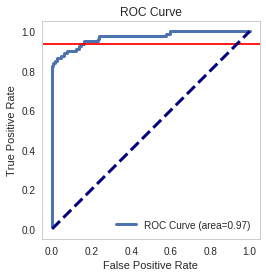index = numpy.where(numpy.round(false_positive_rate, 2)==0.16)
print("True positive rate where false positive rate is 0.16: {0}".format(true_positive_rate[index]))

True positive rate where false positive rate is 0.16: 0.9375

def true_positive_where_false(model, threshold):
"""get the true-positive value matching the threshold for false-positive
Args:
model: the model fit to the data with predict_proba method
Return:
float: True Positive rate

System Message: WARNING/2 (<string>, line 466)

Definition list ends without a blank line; unexpected unindent.

""" y_score_lr = model.predict_proba(X_test) false_positive_rate, true_positive_rate, _ = roc_curve(y_test, y_score_lr[:, 1]) index = numpy.where(numpy.round(false_positive_rate, 2)==0.16) return true_positive_rate[index]

def recall_where_precision(model, threshold):
"""return recall where the first precision matches threshold
Args:
model: model fit to the data with decision_function threshold (float): point to find matching recall
Returns:
float: recall matching precision threshold

System Message: WARNING/2 (<string>, line 482)

Definition list ends without a blank line; unexpected unindent.

""" y_scores = model.decision_function(X_test) precision, recall, thresholds = precision_recall_curve(y_test, y_scores) return recall[numpy.where(precision==threshold)]

model = LogisticRegression() model.fit(X_train, y_train) recall_score = recall_where_precision(model, 0.75) true_positive = true_positive_where_false(model, threshold=0.16) return (recall_score, true_positive)

parameters = dict(penalty=["l1", "l2"], C=[10**power for power in range(-2, 3)]) model = LogisticRegression()

grid = GridSearchCV(model, parameters, scoring="recall") grid.fit(X_train, y_train)

grid.cv_results_

len(grid.cv_results_["mean_test_score"])

grid.cv_results_ l1 = [grid.cv_results_["mean_test_score"][index] for index in range(0, len(grid.cv_results_['mean_test_score']), 2)] l2 = [grid.cv_results_["mean_test_score"][index] for index in range(1, len(grid.cv_results_["mean_test_score"])+ 1, 2)] l1

l2

parameters = dict(penalty=["l1", "l2"], C=[10**power for power in range(-2, 3)]) model = LogisticRegression() grid = GridSearchCV(model, parameters, scoring="recall") grid.fit(X_train, y_train) l1 = [grid.cv_results_["mean_test_score"][index] for index in range(0, len(grid.cv_results_['mean_test_score']), 2)] l2 = [grid.cv_results_["mean_test_score"][index] for index in range(1, len(grid.cv_results_["mean_test_score"])+ 1, 2)] return numpy.array([l1, l2]).T

def GridSearch_Heatmap(scores):
get_ipython().magic('matplotlib inline') import seaborn as sns import matplotlib.pyplot as plt plt.figure() scores = answer_six() sns.heatmap(scores, xticklabels=['l1','l2'], yticklabels=[0.01, 0.1, 1, 10, 100]) plt.yticks(rotation=0);
if VERBOSE:

# Predicting Cancer (Course 3, Assignment 1)

This assignment uses the Breast Cancer Wisconsin (Diagnostic) Database to create a classifier that can help diagnose patients.

## Imports

import numpy
import pandas


## The data

cancer = load_breast_cancer()


This data set has 569 rows (cases) with 30 numeric features. The outcomes are either 1 - malignant, or 0 - benign.

From their description:

Features are computed from a digitized image of a fine needle aspirate (FNA) of a breast mass.  They describe characteristics of the cell nuclei present in the image.


The object returned by load_breast_cancer() is a scikit-learn Bunch object, which is similar to a dictionary, but like pandas, also supports using dot-notation to retrieve attributes when possible (i.e. no spaces in the keys).

print(cancer.keys())

dict_keys(['DESCR', 'target', 'feature_names', 'data', 'target_names'])


## Question 0 (Example)

How many features does the breast cancer dataset have?

def answer_zero():
"""number of feature names in the data

Returns:
int: count of feature names in the 'cancer' data-set
"""
return len(cancer['feature_names'])

answer_zero()

30


## Question 1

Scikit-learn works with lists, numpy arrays, scipy-sparse matrices, and pandas DataFrames, so converting the dataset to a DataFrame is not necessary for training this model. Using a DataFrame does however help make many things easier such as munging data, so let's practice creating a classifier with a pandas DataFrame.

def answer_one():
"""converts the sklearn 'cancer' bunch

Returns:
pandas.DataFrame: cancer data
"""
data = numpy.c_[cancer.data, cancer.target]
columns = numpy.append(cancer.feature_names, ["target"])
return pandas.DataFrame(data, columns=columns)

frame = answer_one()
assert frame.shape == (len(cancer.target), 31)


## Question 2

What is the class distribution? (i.e. how many instances of malignant and how many benign?)

def answer_two():
"""calculates number of malignent and benign

Returns:
pandas.Series: counts of each
"""
counts = cancerdf.target.value_counts(ascending=True)
counts.index = "malignant benign".split()
return counts

output = answer_two()
assert output.malignant == 212
assert output.benign == 357


## Question 3

Split the DataFrame into X (the data) and y (the labels).

def answer_three():
"""splits the data into data and labels

Returns:
(pandas.DataFrame, pandas.Series): data, labels
"""
X = cancerdf[cancerdf.columns[:-1]]
y = cancerdf.target
return X, y

x, y = answer_three()
assert x.shape == (569, 30)
assert y.shape == (569,)


## Question 4

Using train_test_split(), split X and y into training and test sets (X_train, X_test, y_train, and y_test).

from sklearn.model_selection import train_test_split

"""splits data into training and testing sets

Returns:
tuple(pandas.DataFrame): x_train, y_train, x_test, y_test
"""
return train_test_split(X, y, train_size=426, test_size=143, random_state=0)

x_train, x_test, y_train, y_test = answer_four()
assert x_train.shape == (426, 30)
assert x_test.shape == (143, 30)
assert y_train.shape == (426,)
assert y_test.shape == (143,)


## Question 5

Using KNeighborsClassifier, fit a k-nearest neighbors (knn) classifier with X_train, y_train and using one nearest neighbor (n_neighbors = 1).

from sklearn.neighbors import KNeighborsClassifier

"""Fits a KNN-1 model to the data

Returns:
sklearn.neighbors.KNeighborsClassifier: trained data
"""
X_train, X_test, y_train, y_test = answer_four()
model = KNeighborsClassifier(n_neighbors=1)
model.fit(X_train, y_train)
return model

knn = answer_five()
assert type(knn) == KNeighborsClassifier
assert knn.n_neighbors == 1


## Question 6

Using your knn classifier, predict the class label using the mean value for each feature.

You can use cancerdf.mean()[:-1].values.reshape(1, -1) which gets the mean value for each feature, ignores the target column, and reshapes the data from 1 dimension to 2 (necessary for the predict method of KNeighborsClassifier).

def answer_six():
"""Predicts the class labels for the means of all features

Returns:
numpy.array: prediction (0 or 1)
"""
means = cancerdf.mean()[:-1].values.reshape(1, -1)
return model.predict(means)

answer_six()

array([ 1.])


## Question 7

Using your knn classifier, predict the class labels for the test set X_test.

def answer_seven():
"""predicts likelihood of cancer for test set

Returns:
numpy.array: vector of predictions
"""
X_train, X_test, y_train, y_test = answer_four()
return knn.predict(X_test)

predictions = answer_seven()
assert predictions.shape == (143,)
assert set(predictions) == {0.0, 1.0}

print("no cancer: {0}".format(len(predictions[predictions==0])))
print("cancer: {0}".format(len(predictions[predictions==1])))


## Question 8

Find the score (mean accuracy) of your knn classifier using X_test and y_test.

def answer_eight():
"""calculates the mean accuracy of the KNN model

Returns:
float: mean accuracy of the model predicting cancer
"""
X_train, X_test, y_train, y_test = answer_four()
return knn.score(X_test, y_test)

answer_eight()


## Optional plot

Try using the plotting function below to visualize the differet predicition scores between training and test sets, as well as malignant and benign cells.

%matplotlib inline

def accuracy_plot():
import matplotlib.pyplot as plt

X_train, X_test, y_train, y_test = answer_four()

# Find the training and testing accuracies by target value (i.e. malignant, benign)
mal_train_X = X_train[y_train==0]
mal_train_y = y_train[y_train==0]
ben_train_X = X_train[y_train==1]
ben_train_y = y_train[y_train==1]

mal_test_X = X_test[y_test==0]
mal_test_y = y_test[y_test==0]
ben_test_X = X_test[y_test==1]
ben_test_y = y_test[y_test==1]

scores = [knn.score(mal_train_X, mal_train_y), knn.score(ben_train_X, ben_train_y),
knn.score(mal_test_X, mal_test_y), knn.score(ben_test_X, ben_test_y)]

plt.figure()

# Plot the scores as a bar chart
bars = plt.bar(numpy.arange(4), scores, color=['#4c72b0','#4c72b0','#55a868','#55a868'])

# directly label the score onto the bars
for bar in bars:
height = bar.get_height()
plt.gca().text(bar.get_x() + bar.get_width()/2, height*.90, '{0:.{1}f}'.format(height, 2),
ha='center', color='w', fontsize=11)

# remove all the ticks (both axes), and tick labels on the Y axis
plt.tick_params(top='off', bottom='off', left='off', right='off', labelleft='off', labelbottom='on')

# remove the frame of the chart
for spine in plt.gca().spines.values():
spine.set_visible(False)

plt.xticks([0,1,2,3], ['Malignant\nTraining', 'Benign\nTraining', 'Malignant\nTest', 'Benign\nTest'], alpha=0.8);
plt.title('Training and Test Accuracies for Malignant and Benign Cells', alpha=0.8)
accuracy_plot()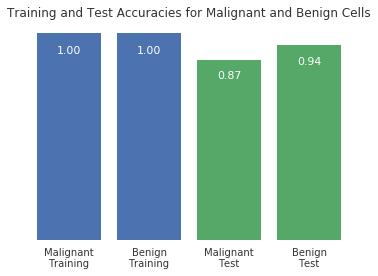# Bokeh Test

## What This Is

This is a re-do of the final plot done for data-science with python course 2 week 4. The original was done with matplotlib and this was done with bokeh to get some interaction working. When I tried to create it the first time bokeh raised some errors saying that height had been defined more than once. I don't know what really caused it - possibly a namespace clash where I was re-using something I didn't intend to re-use - but when I created a new notebook that only created the one plot it worked. Since this uses javascript I used Jupyter and the web-inteface to test it out (emacs ipython doesn't seem to be able to render javascript (unless I'm doing it wrong)).

## How It Got Exported

I won't go over the creating of the data (since I just copied it from an earlier notebook) but this is how the bokeh plot was created.

### Imports

These were the bokeh parts I needed.

from bokeh.models import (
BoxAnnotation,
CustomJS,
Span,
Toggle,
)

from bokeh.io import (
output_file,
output_notebook,
show,
)

from bokeh.plotting import (
figure,
ColumnDataSource,
)

from bokeh.models import (
CrosshairTool,
HoverTool,
PanTool,
ResetTool,
ResizeTool,
SaveTool,
UndoTool,
WheelZoomTool,
)

from bokeh.layouts import column
from bokeh.resources import CDN


### Some Constants

NATIONAL_COLOR = "slategrey"
NATIONAL_LABEL = "National"
PORTLAND_COLOR = "cornflowerblue"
PORTLAND_LABEL = "Portland-Hillsboro-Vancouver"
S_AND_P_COLOR = "#90151B"
S_AND_P_LABEL = "S & P 500 Index"
HOUSING_COLOR = "#D89159"
HOUSING_LABEL = "House Price Index"


### The Data

bokeh doesn't work with pandas DataFrame's (or at least I couldn't get it to work). Instead you create a DataFrame-like object using the ColumnDataSource.

portland_source = ColumnDataSource(
data=dict(
month_data=portland.datetime,
unemployment=portland.unemployment_rate,
month_label=portland.date,
)
)

national_source = ColumnDataSource(
data=dict(
month_data=national.datetime,
unemployment=national.unemployment_rate,
month_label=national.date,
)
)

housing_source = ColumnDataSource(
data=dict(
month_data=house_price_index.datetime,
price=house_price_index.price,
month_label=s_and_p_index.date,
)
)

s_and_p_source = ColumnDataSource(
data=dict(
month_data=s_and_p_index.datetime,
value=s_and_p_index.VALUE,
month_label=s_and_p_index.date,
)
)


### The Tools

These are the things that add interactivity to the plot. You have to create new ones for each figure so I made a function to get them.

def make_tools():
"""makes the tools for the figures

Returns:
list: tool objects
"""
hover = HoverTool(tooltips=[
("month", "@month_label"),
("unemployment", "@unemployment"),
])

tools = [
hover,
CrosshairTool(),
PanTool(),
ResetTool(),
ResizeTool(),
SaveTool(),
UndoTool(),
WheelZoomTool(),
]


The HoverTool tooltips argument is a list of tuples - one tuple for each dimension of the data. The first argument of the tuple (e.g. "month") is the label that will appear when the user hover's over the data point, while the second (e.g. "@month_label") tells bokeh which column to use for the data (so it has to match the key you used in the ColumnDataSource creation).

### Helper Functions

The sub-figures needed some common elements so I created functions for them.

#### Scaling The Timestamps

The timestamps by default are unreadable (because there are so many). This re-scales them so they are more readable.

def scale_timestamp(index):
"""gets the scaled timestamp for element location

Args:
index: index in the portland.datetime series
Returns:
epoch timestamp used to locate place in plot
"""
return portland.datetime[index].timestamp() * TIME_SCALE


#### Drawing the Recession

The recession is indicated as a blue box on each plot.

def make_recession():
"""Makes the box for the recession

Returns:
BoxAnnotation to color the recession
"""
return BoxAnnotation(
left=scale_timestamp(recession_start),
right=scale_timestamp(recession_end),
fill_color="blue",
fill_alpha=0.1)


#### Vertical Lines

Things like the unemployment lows and highs are indicated by a vertical line.

def make_vertical(location, color="darkorange"):
"""makes a vertical line

Args:
location: place on the x-axis for the line
color (str): line-color for the line
Returns:
Span at index
"""
return Span(
location=location,
line_color=color,
dimension="height",
)


#### Make Verticals

Since there's more than one line, this function adds all the lines.

def make_verticals(fig):
"""makes the verticals and adds them to the figures"""
location=scale_timestamp(unemployment_peaks),
color="darkorange",
))
color="crimson"))
color="limegreen"))
location=scale_timestamp(national_peak),
color="grey"))
return


### The Figures

This plot has three sub-figures, each of which is created separately then added to the Column.

#### Unemployment

tools = make_tools()
unemployment_figure = figure(
plot_width=FIGURE_WIDTH,
plot_height=FIGURE_HEIGHT,
x_axis_type="datetime",
tools=tools,
title="Portland Unemployment (2007-2017)"
)


Next the lines for the time-series data are added.

unemployment_figure.line(
"month_data", "unemployment",
source=portland_source,
line_color=PORTLAND_COLOR,
legend=PORTLAND_LABEL,
)

line = unemployment_figure.line(
"month_data", "unemployment",
source=national_source,
line_color=NATIONAL_COLOR,
legend=NATIONAL_LABEL,
)


Now the recession-box and high and low points for each plot is added.

unemployment_figure.add_layout(make_recession())
make_verticals(unemployment_figure)


Now some labels are added and the grid is turned off.

unemployment_figure.yaxis.axis_label = "% Unemployment"
unemployment_figure.xaxis.axis_label = "Month"
unemployment_figure.xgrid.visible = False
unemployment_figure.ygrid.visible = False


#### S & P 500

The S & P 500 had didn't have unemployment as the dependent variable so I made a different set of tools to change the label for the hover.

hover = HoverTool(tooltips=[
("Month", "@month_label"),
("Value", "@value"),
])
tools = [
hover,
CrosshairTool(),
PanTool(),
ResetTool(),
ResizeTool(),
SaveTool(),
UndoTool(),
WheelZoomTool(),
]
s_and_p_figure = figure(
plot_width=FIGURE_WIDTH,
plot_height=FIGURE_HEIGHT,
x_range=unemployment_figure.x_range,
x_axis_type="datetime",
tools=tools,
title="S & P 500 Index",
)
line = s_and_p_figure.line("month_data", "value",
source=s_and_p_source,
line_color=S_AND_P_COLOR)
make_verticals(s_and_p_figure)
s_and_p_figure.yaxis.axis_label = "S & P 500 Valuation"
s_and_p_figure.xaxis.axis_label = "Month"
s_and_p_figure.xgrid.visible = False
s_and_p_figure.ygrid.visible = False
s_and_p_figure.legend.location = "bottom_right"


#### Housing

hover = HoverTool(tooltips=[
("Month", "@month_label"),
("Price", "@price"),
])
tools = [
hover,
CrosshairTool(),
PanTool(),
ResetTool(),
ResizeTool(),
SaveTool(),
UndoTool(),
WheelZoomTool(),
]
housing_figure = figure(
plot_width=FIGURE_WIDTH,
plot_height=FIGURE_HEIGHT,
x_range=unemployment_figure.x_range,
x_axis_type="datetime",
tools=tools,
title="House Price Index",
)
line = housing_figure.line("month_data", "price",
source=housing_source,
line_color=HOUSING_COLOR)
make_verticals(housing_figure)
housing_figure.yaxis.axis_label = "Sale Price (\$1,000)"
housing_figure.xaxis.axis_label = "Month"
housing_figure.xgrid.visible = False
housing_figure.ygrid.visible = False
housing_figure.legend.location = "bottom_right"


### Combining

Once the figures were created I combined them into a column, since I wanted them stacked verticallly.

combined = column(unemployment_figure, s_and_p_figure, housing_figure)


### Outputting The Code

In order to be able to embed the code, you need to have bokeh export it. There are multiple ways to do this, but I chose the autoload_static method.

OUTPUT_JAVASCRIPT = "portland_unemployment.js"
js, tag = autoload_static(combined, CDN, OUTPUT_JAVASCRIPT)


The third argument (OUTPUT_JAVASCRIPT) is the path you want to refer to in the tag. The returned js variable contains the javascript you need to save (using the filename you gave autoload_static) and the tag contains the HTML tag that you embed to let the server know you want to use the javascript that was saved.

Since both values are just strings, and nothing was saved to disk, I saved it for later.

with open(OUTPUT_JAVASCRIPT, "w") as writer:
writer.write(js)

with open("portland_tag.html", 'w') as writer:
writer.write(tag)


### Getting It Into Nikola

The first thing was to create this file using nikola new_post (it's called bokeh-test.rst). Next I created a directory in the files folder that had the same name as this file (without the ".rst" extension) to put the javascript in so nikola would find it when I built the HTML.

mkdir files/posts/bokeh-test


Once I copied the portland_unemployment.js file to the bokeh-test directory I opened the portland_tag.html file and embedded it directly into the post sing the raw restructureText directive.

.. raw:: html

<script
src="portland_unemployment.js"
id="686c5dd6-168a-4f7d-acbc-524875d93b59"
data-bokeh-model-id="c473232a-dc2c-4b75-988c-f9bc6517b4b9"
data-bokeh-doc-id="402d8e3c-1595-4d65-9f76-e11068c629ab"
></script>